# RD Sharma Solutions for Class 11 Maths Chapter 10 Sine and Cosine Formulae and their Applications

## RD Sharma Solutions Class 11 Maths Chapter 10 – Avail Free PDF Updated Session 2021 – 2022

RD Sharma Solutions for Class 11 Maths Chapter 10 – Sine and Cosine Formulae and their Applications are provided here for students to study and prepare for their board examination. To achieve a good academic score in Mathematics, students are required to practice all the questions of each and every exercise present in the chapter. In this chapter, we shall learn about some trigonometric relations with elements of a triangle. Experts at BYJU’S have formulated the solutions where students can secure good marks in the exams by solving all the questions and cross-checking the answers with the RD Sharma Solutions prepared by the experts in Maths.

RD Sharma Class 11 Solutions for Maths mainly focuses on learning various Mathematical tricks and shortcuts for quick and easy calculations. Students can easily get the pdf of RD Sharma Solutions for Class 11 Maths Chapter 10 Sine and Cosine Formulae and their Applications from the links given below.

Chapter 10 – Sine and Cosine Formulae and their Applications contains two exercises and the RD Sharma Solutions present in this page provide solutions to the questions present in each exercise. Now, let us have a look at the concepts discussed in this chapter.

• The law of sines or sine rule.
• The law of cosines.
• Projection formulae.
• Napier’s analogy (law of tangents).
• Area of a triangle.

## Download the Pdf of RD Sharma Solutions for Class 11 Maths Chapter 10 – Sine and Cosine Formulae and their Applications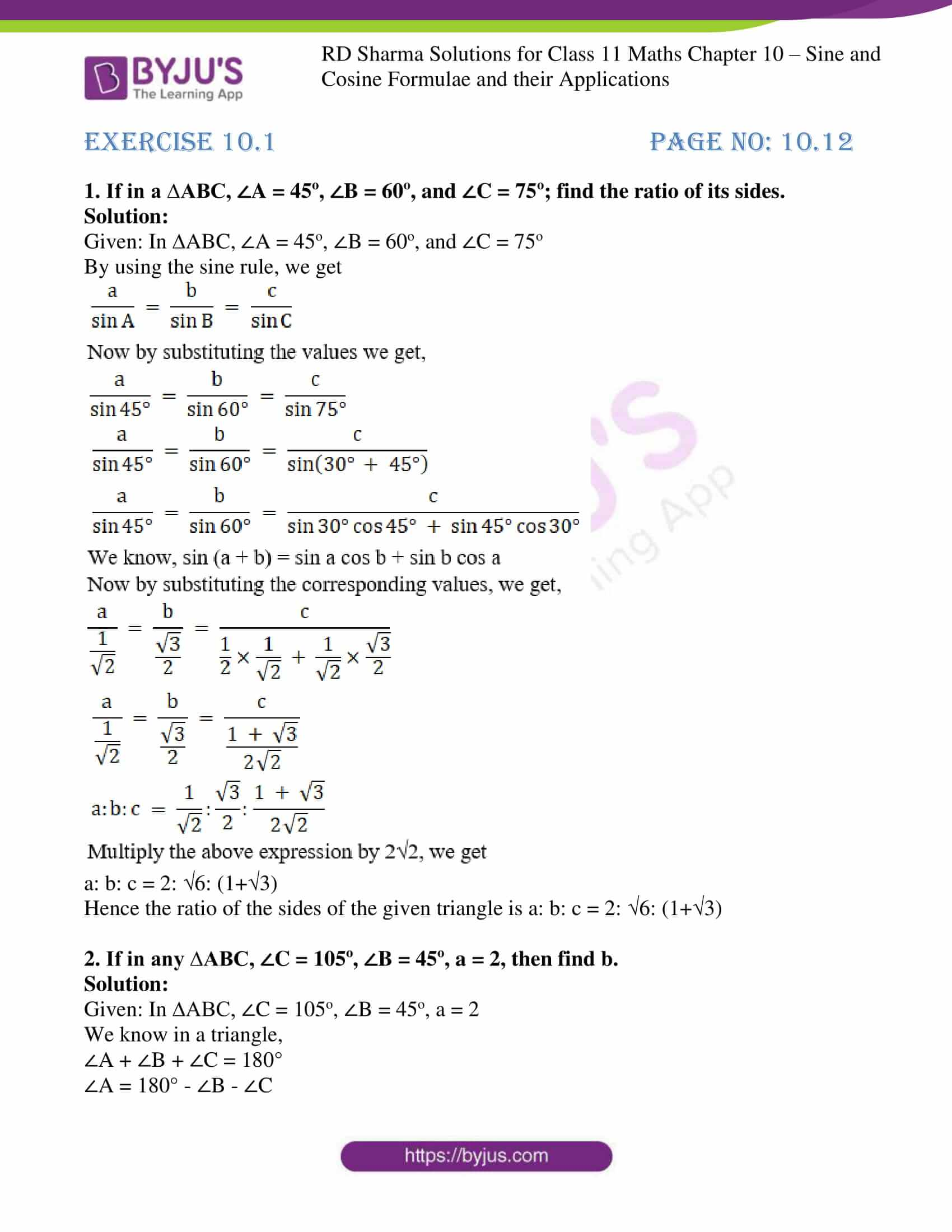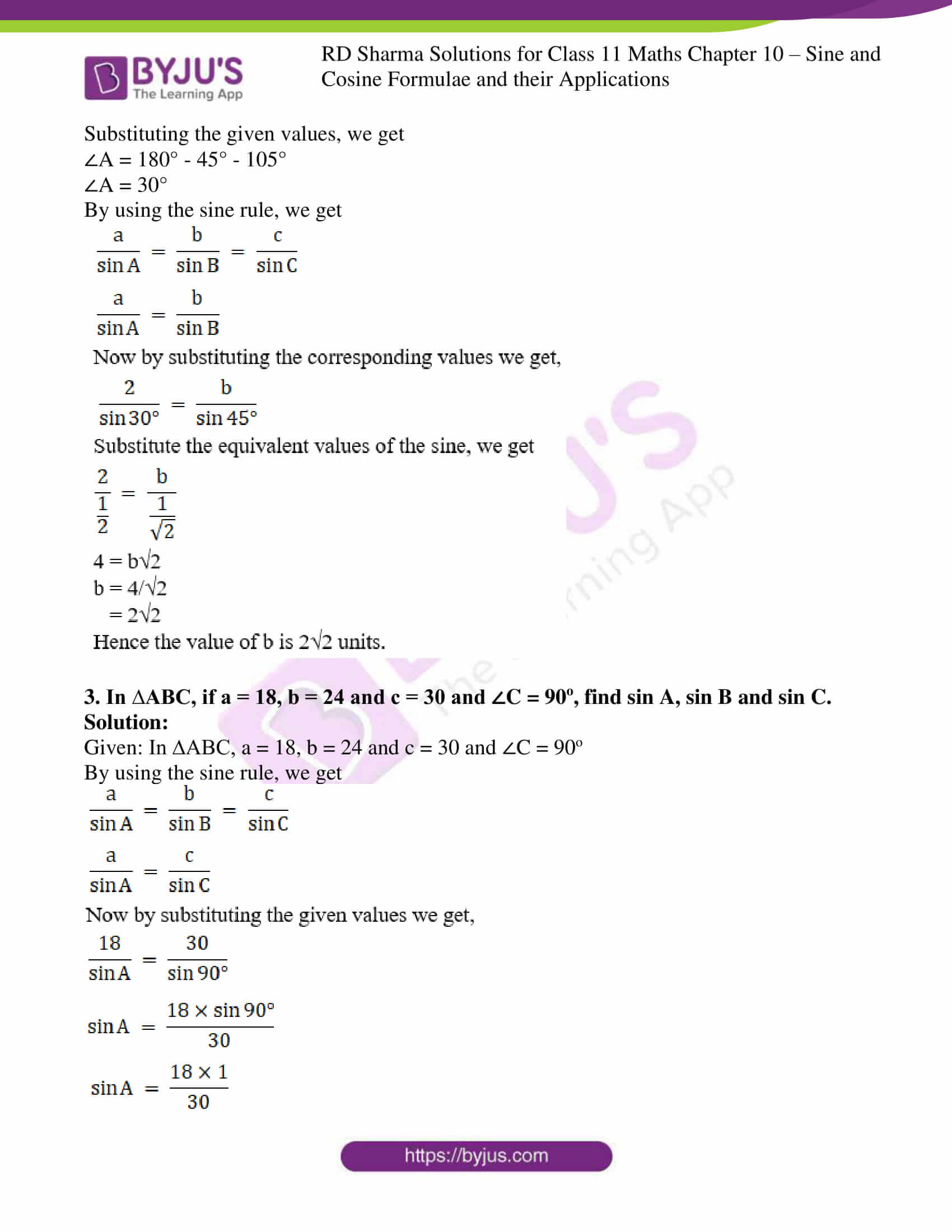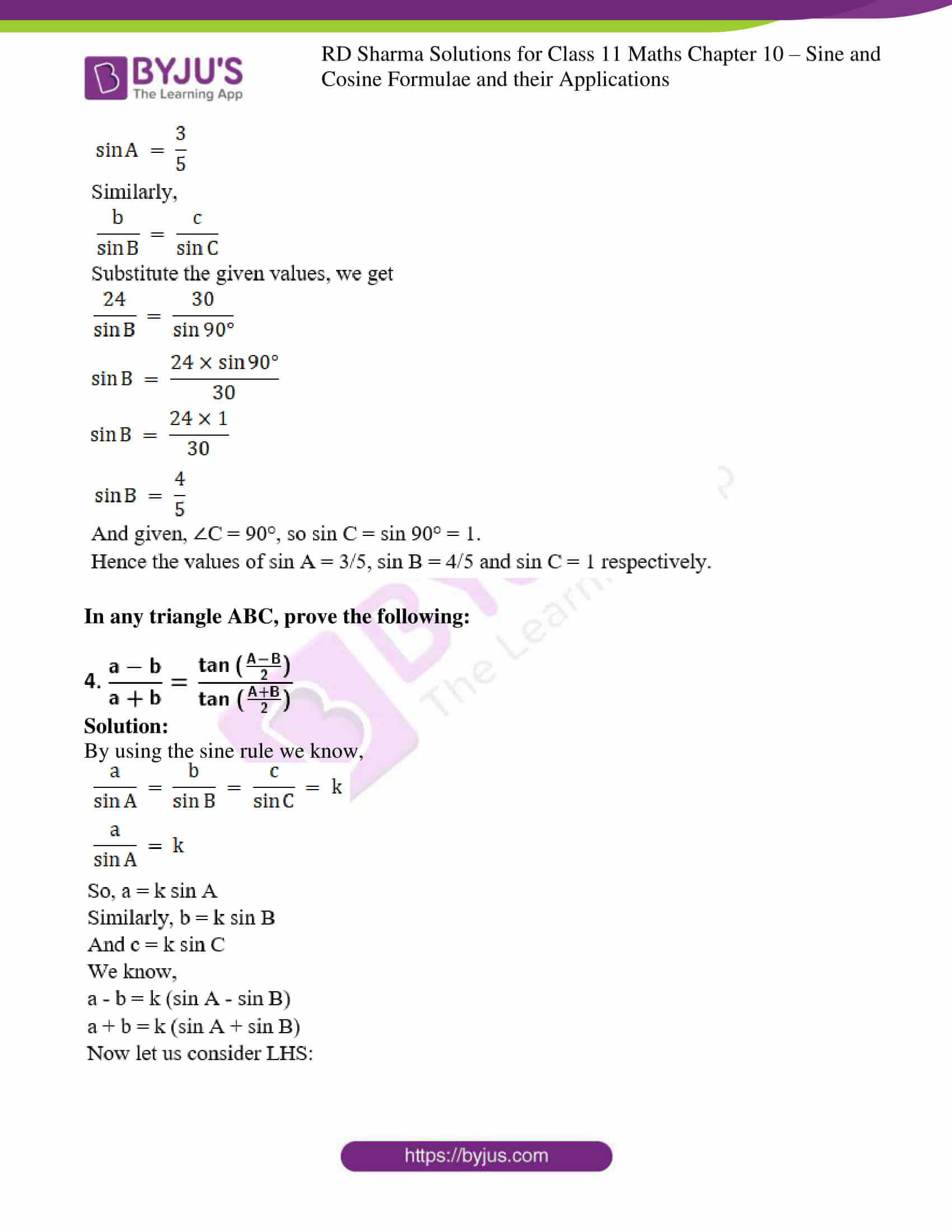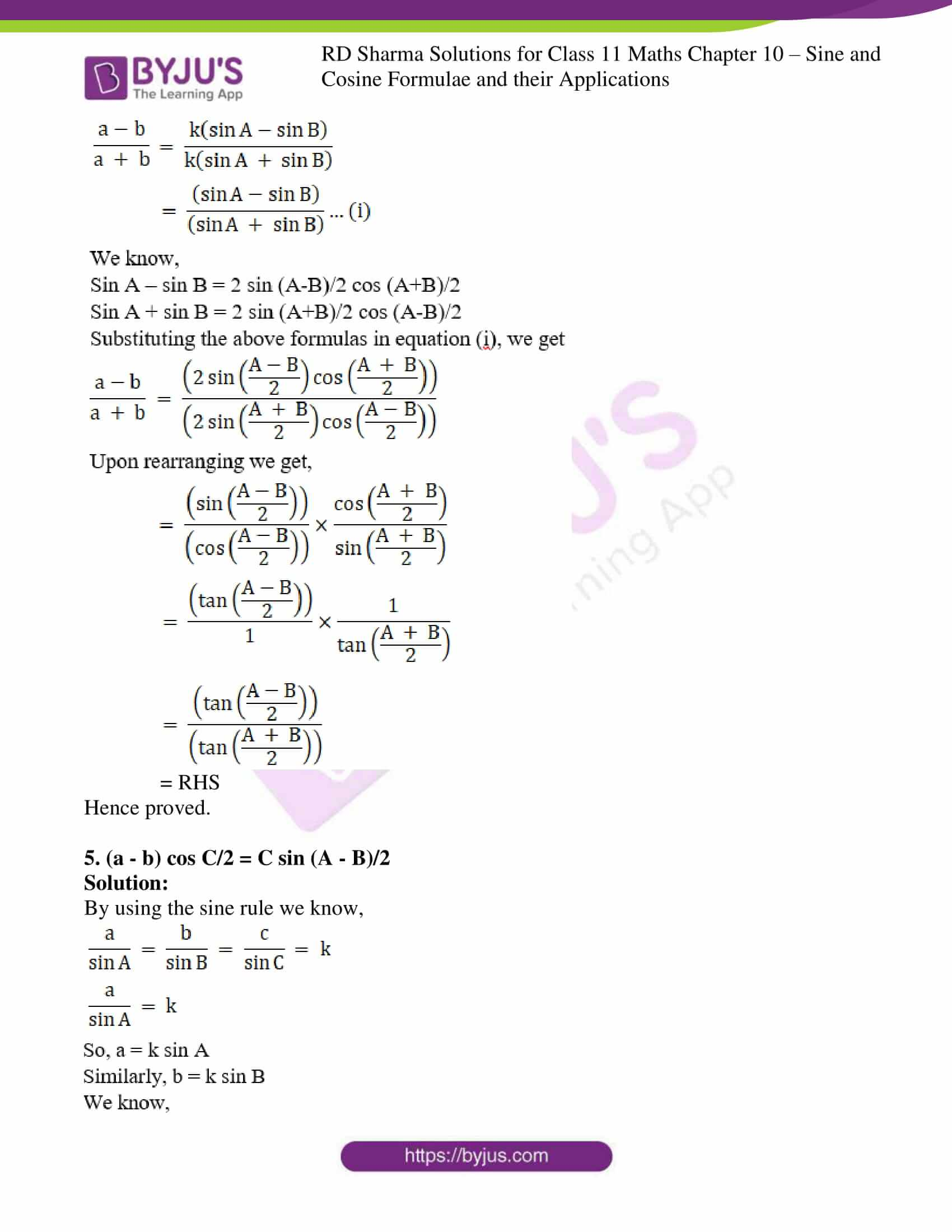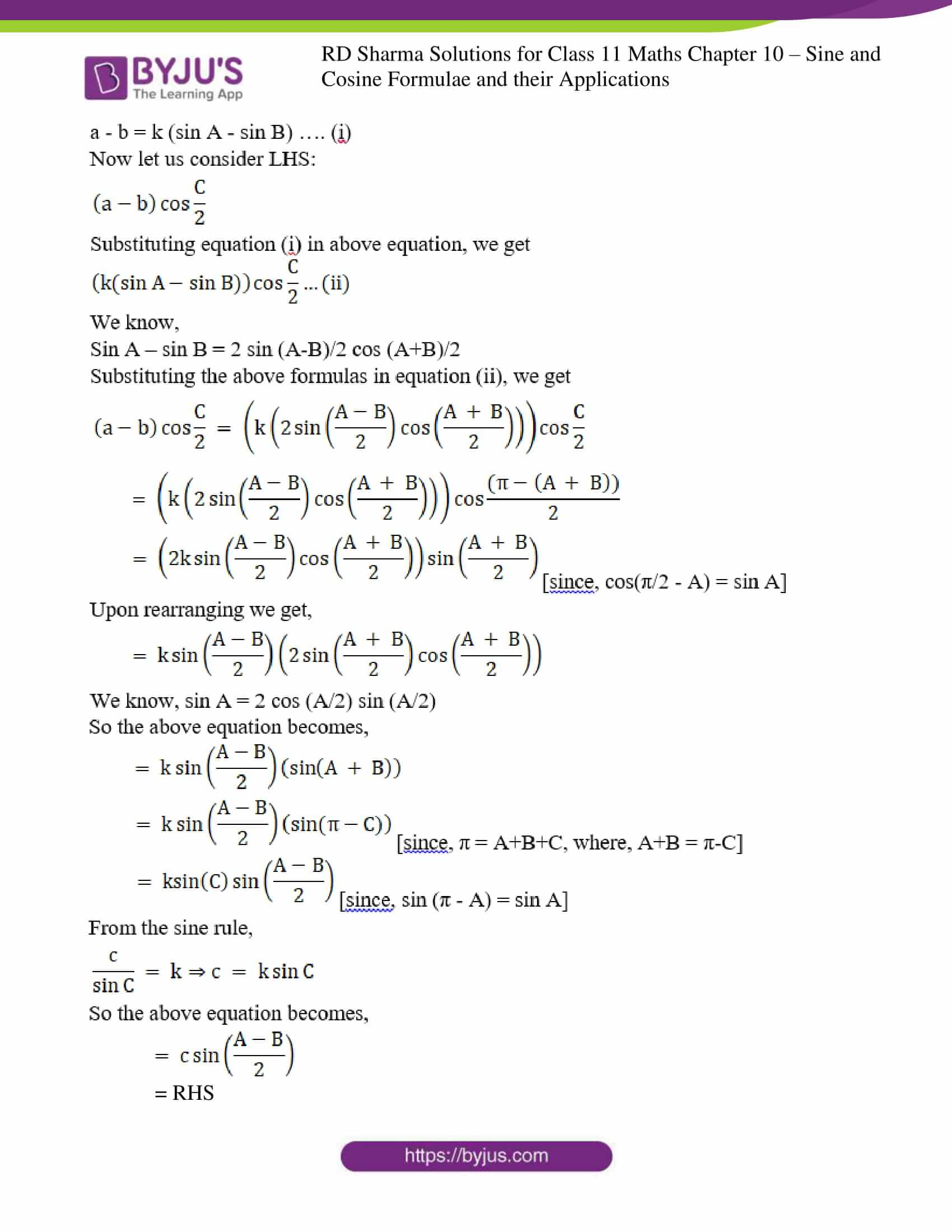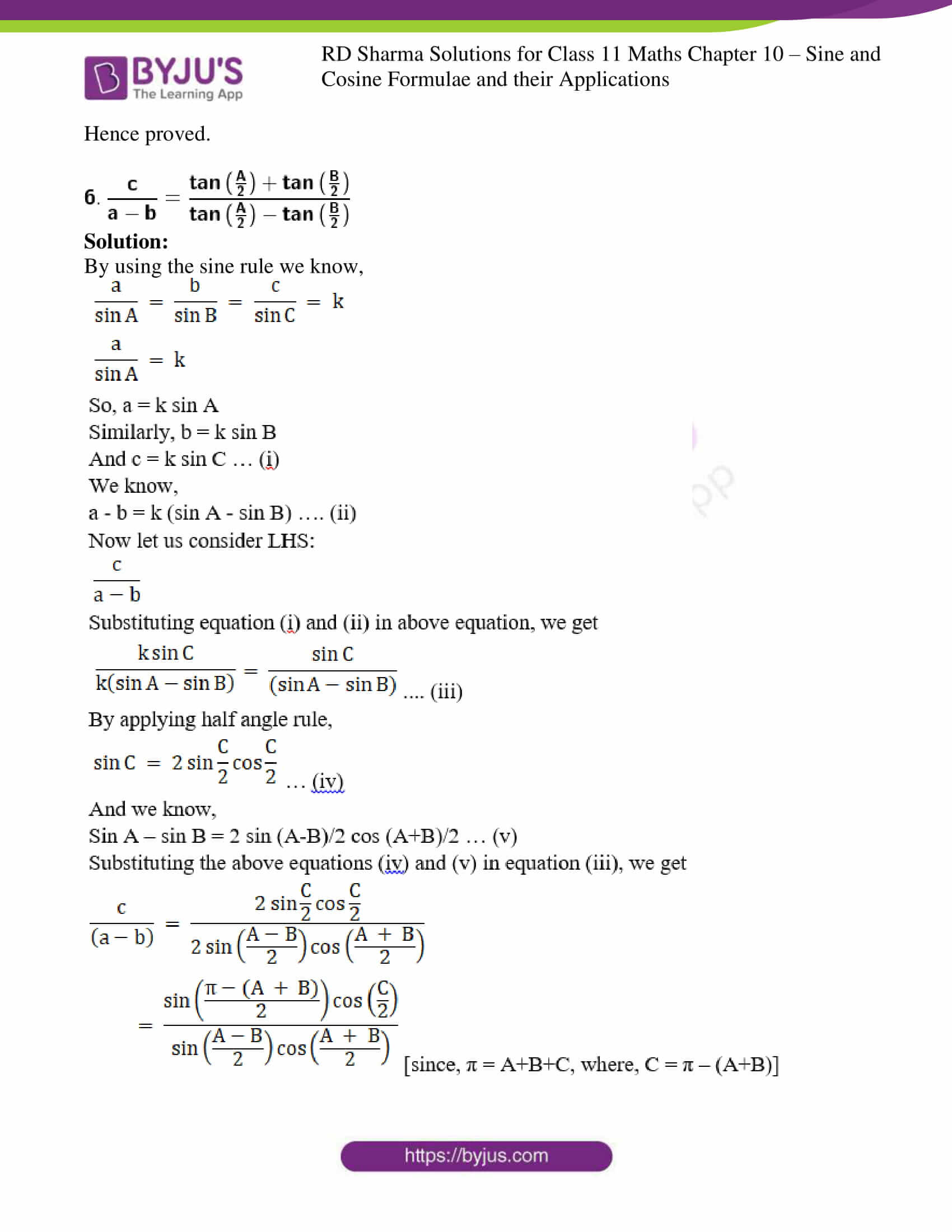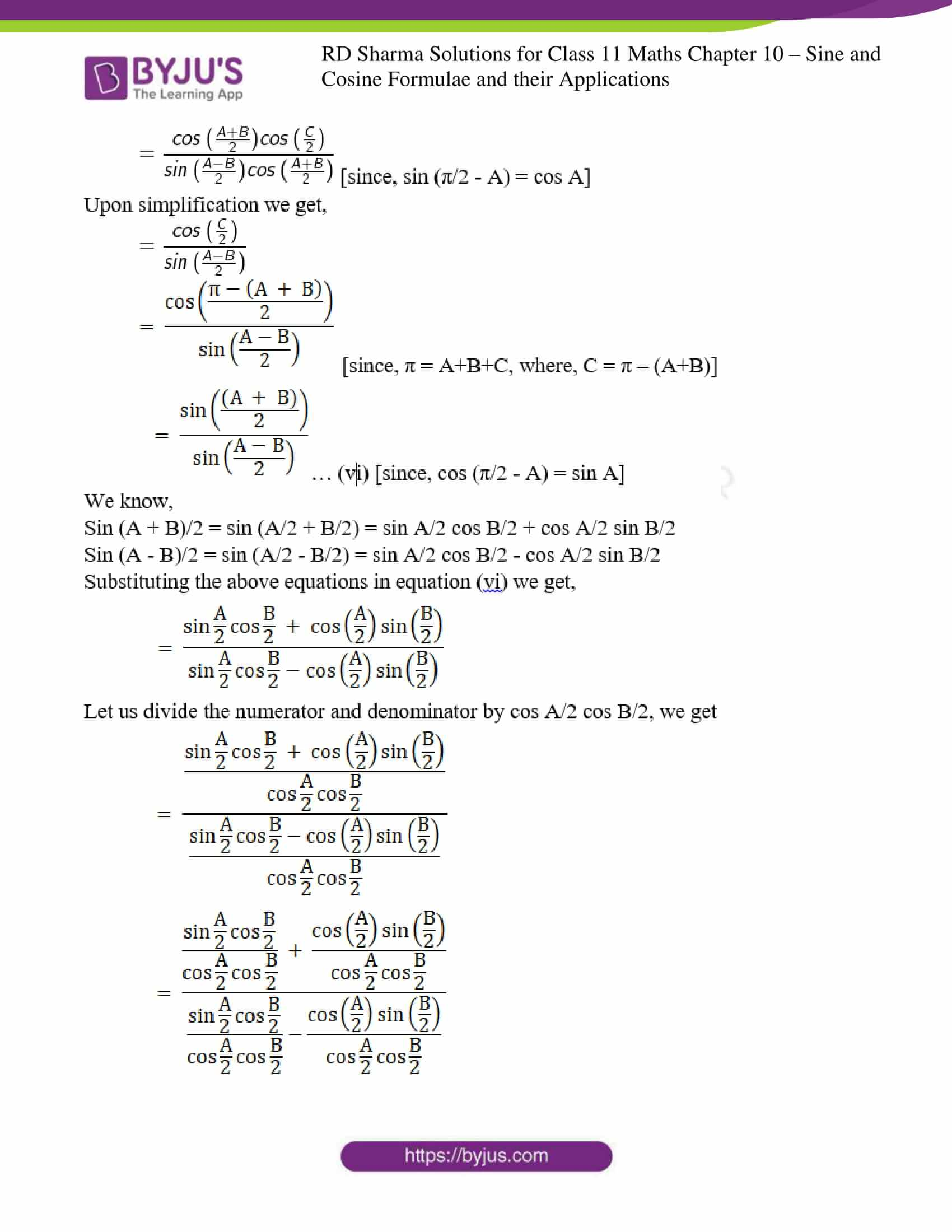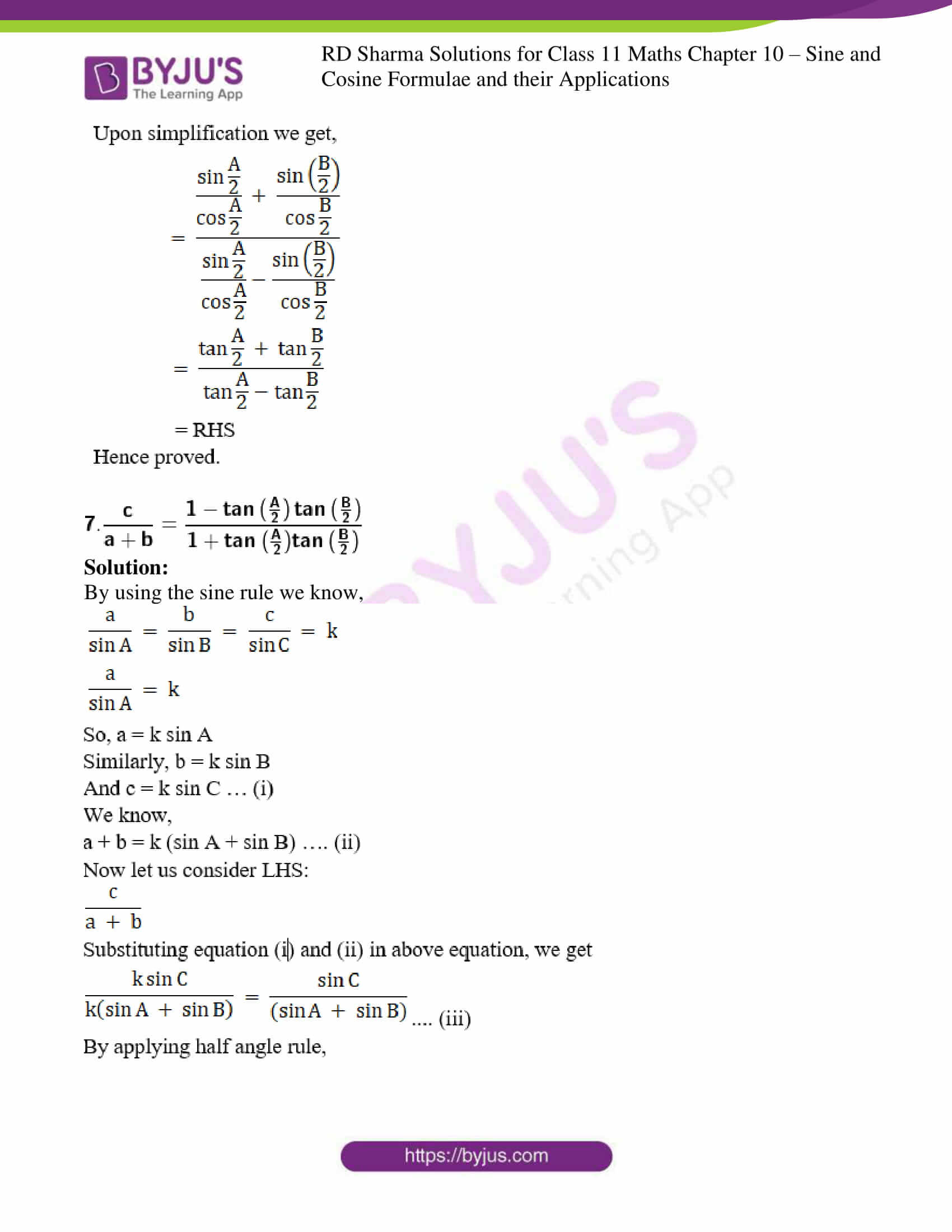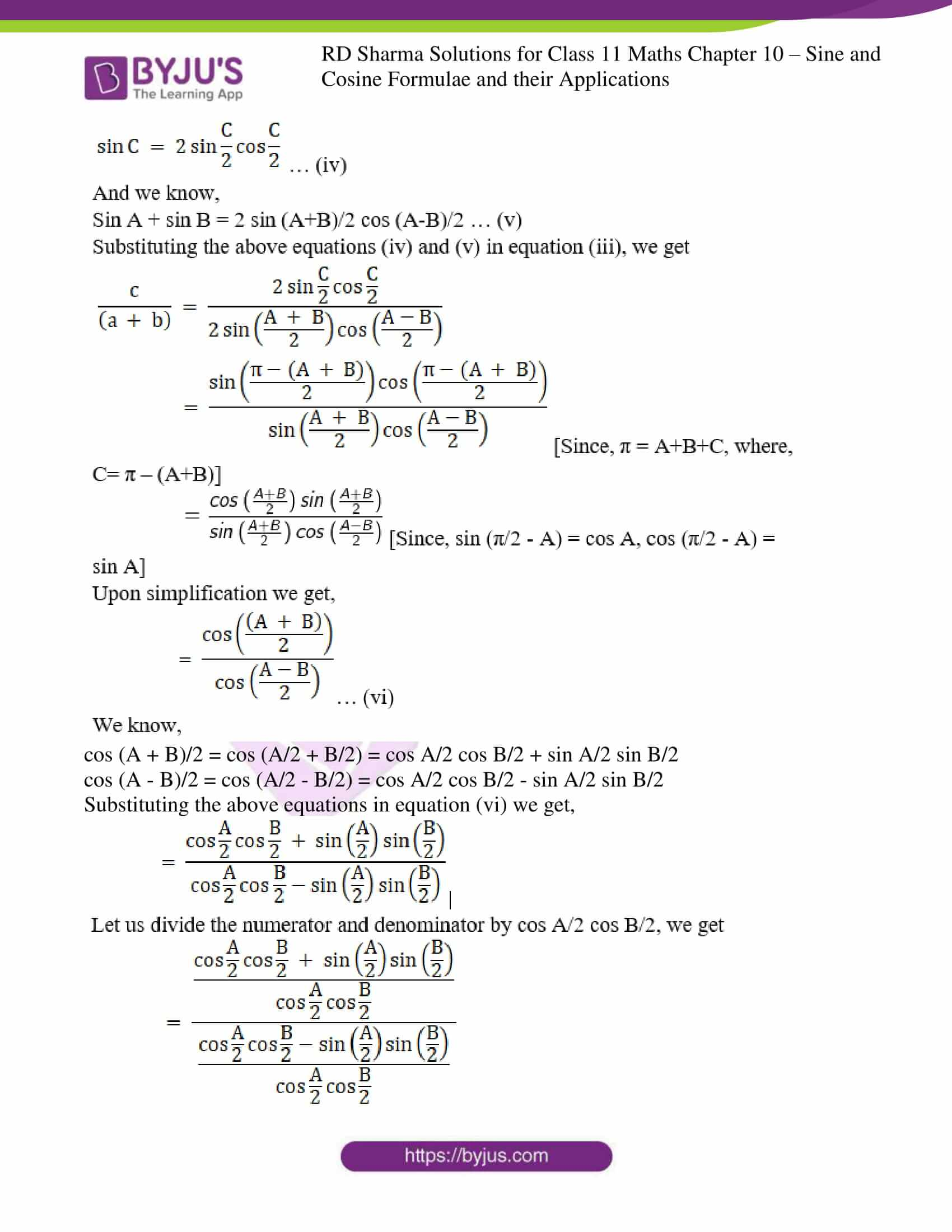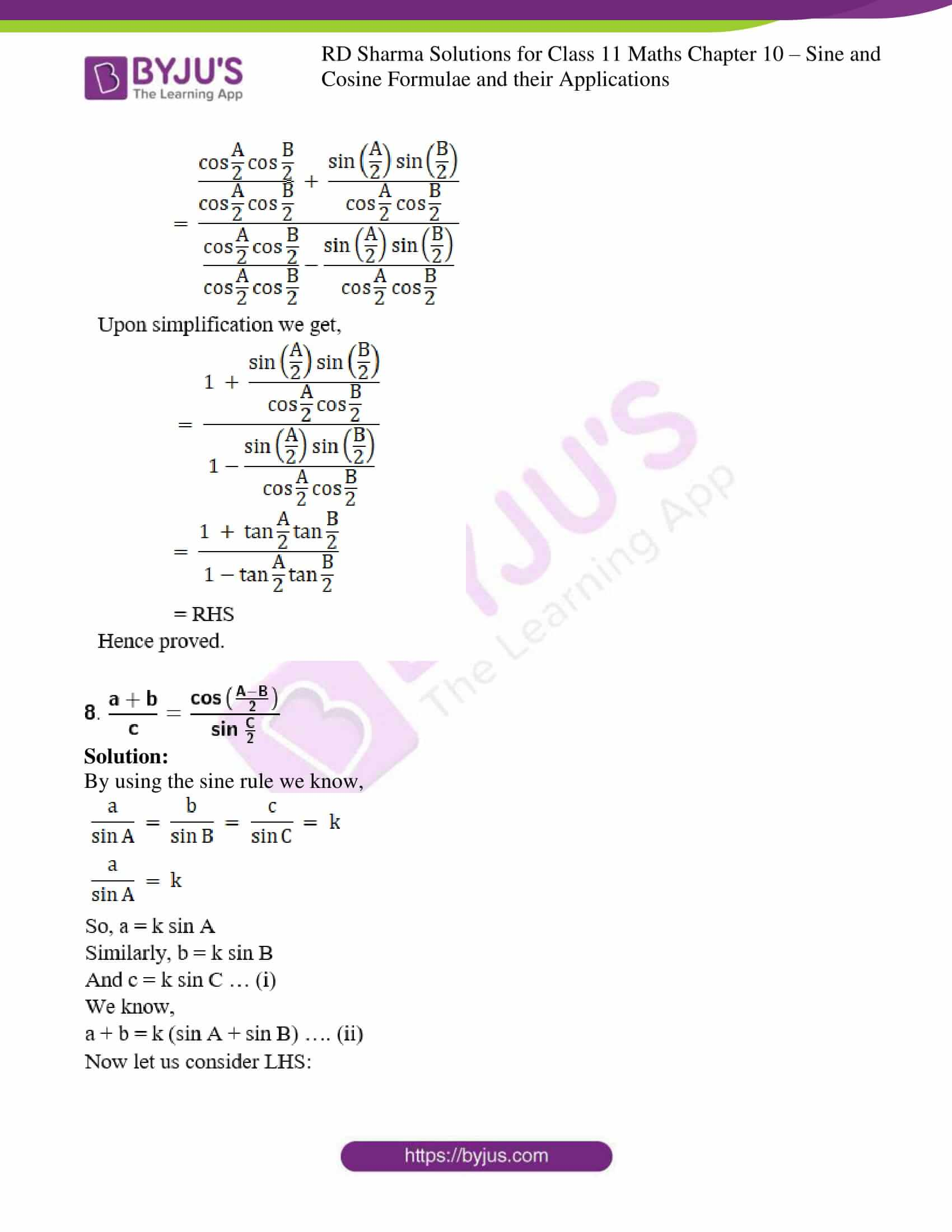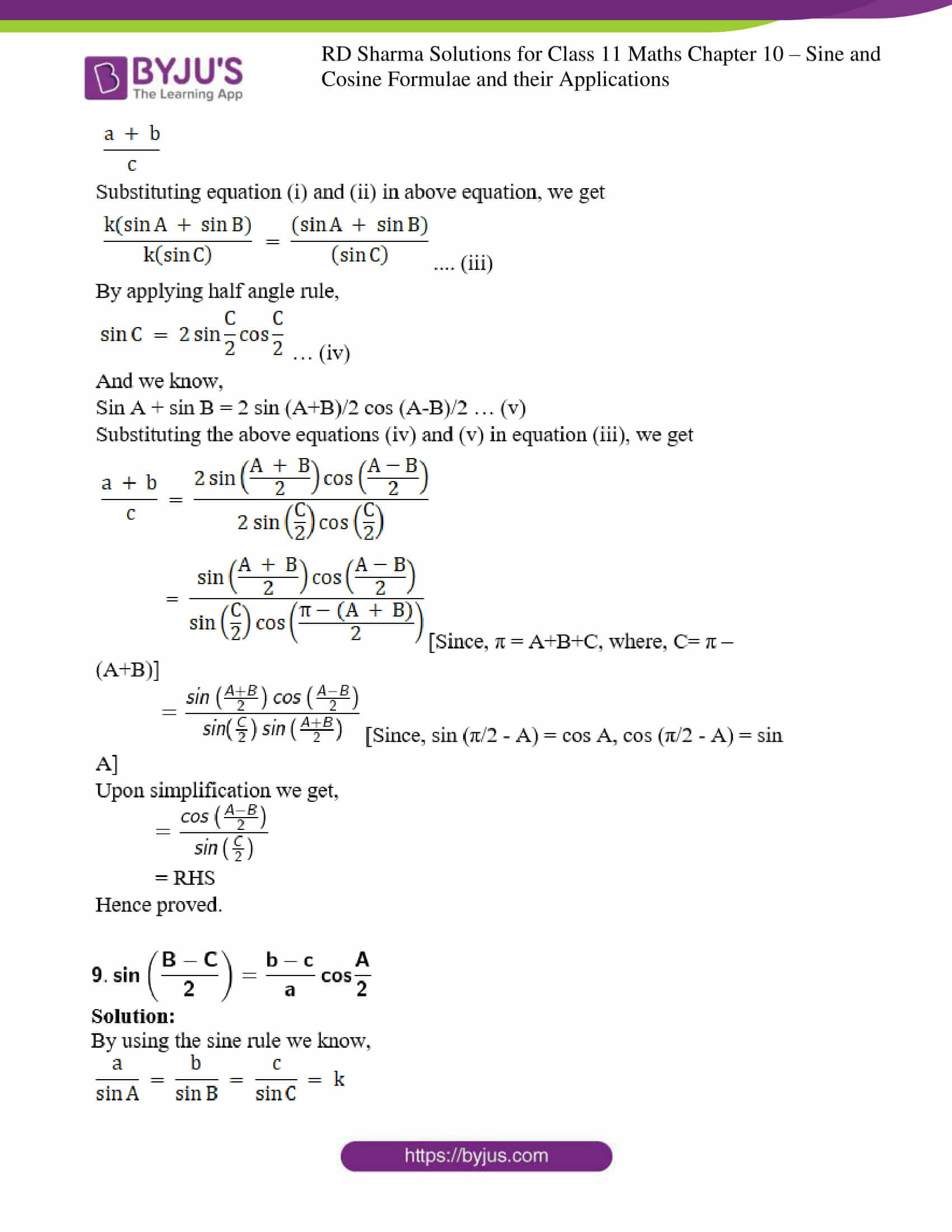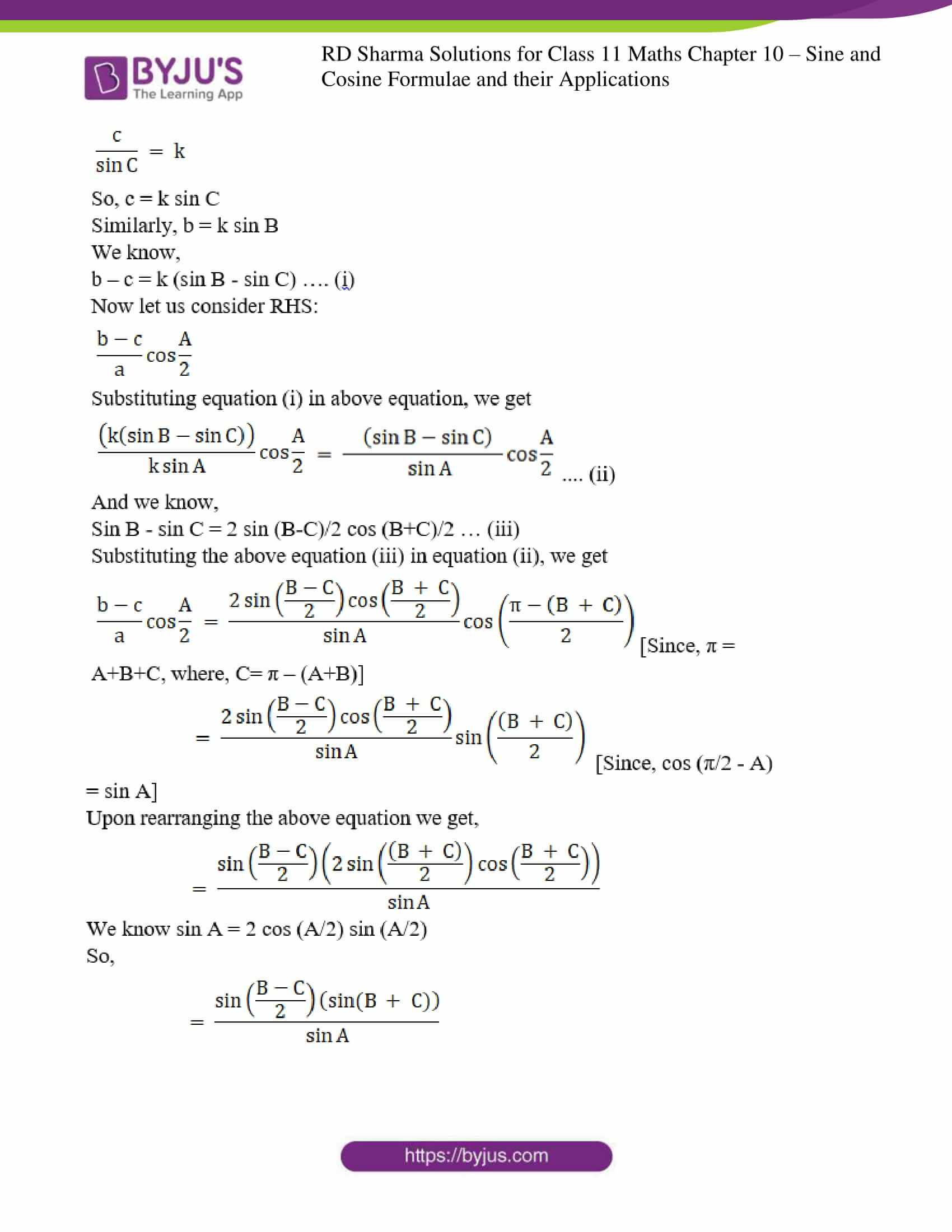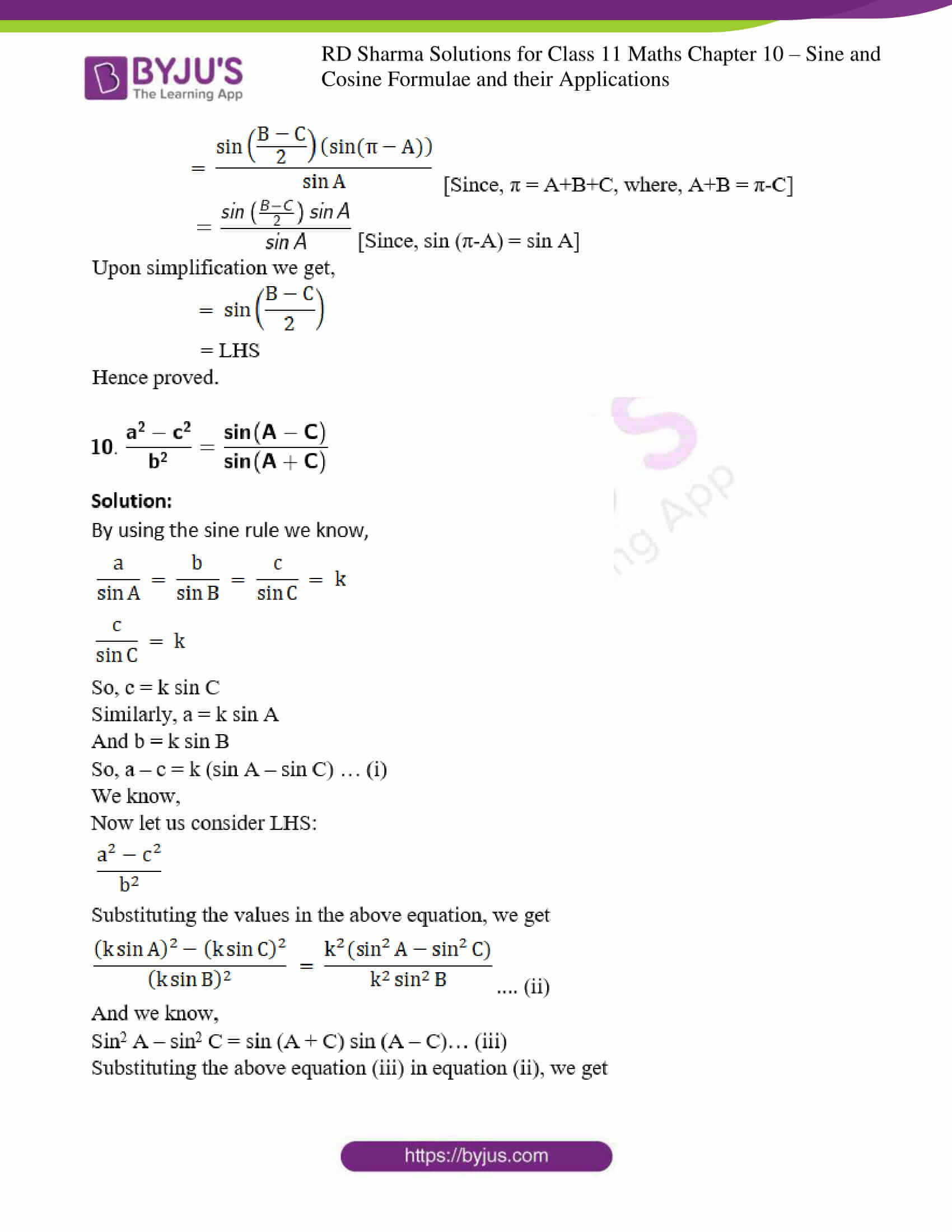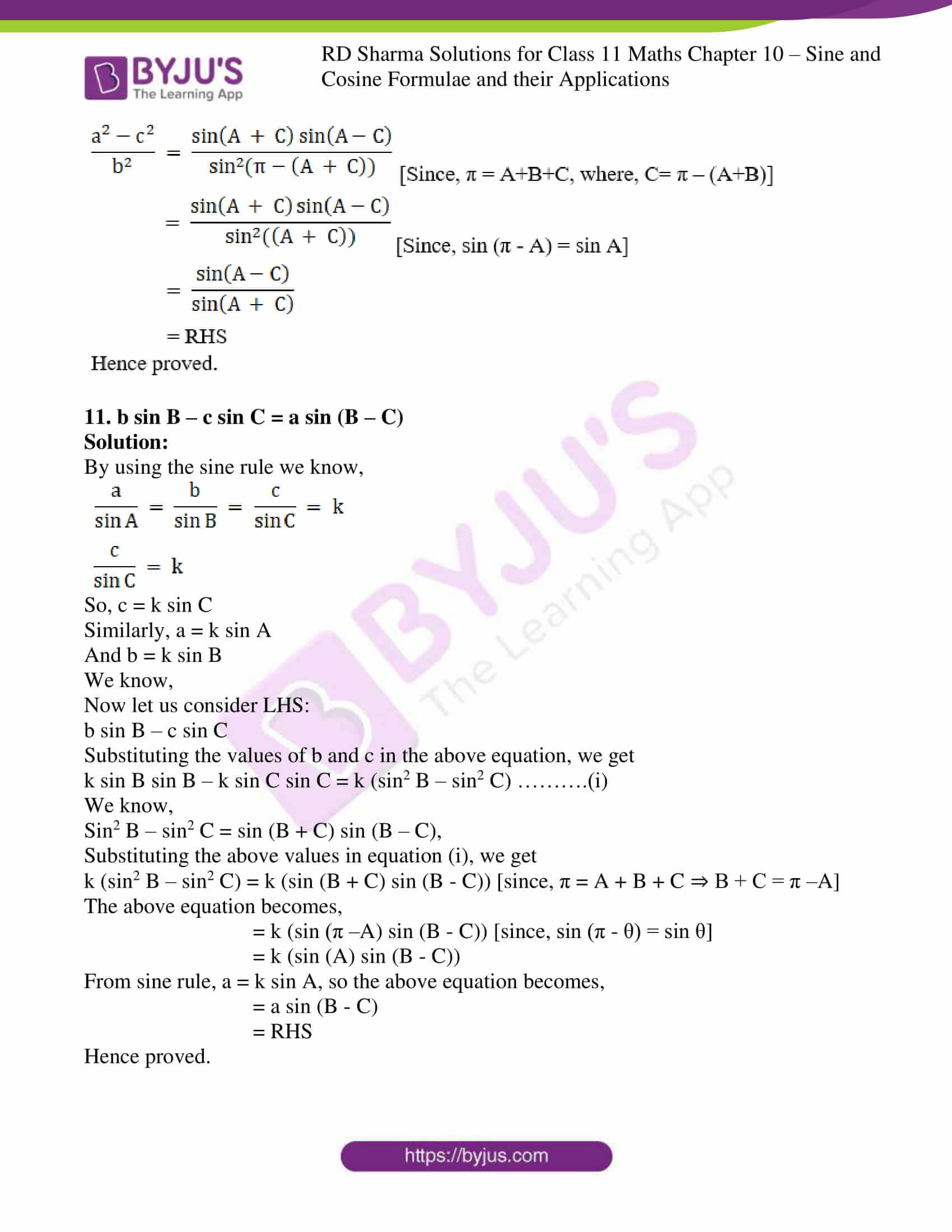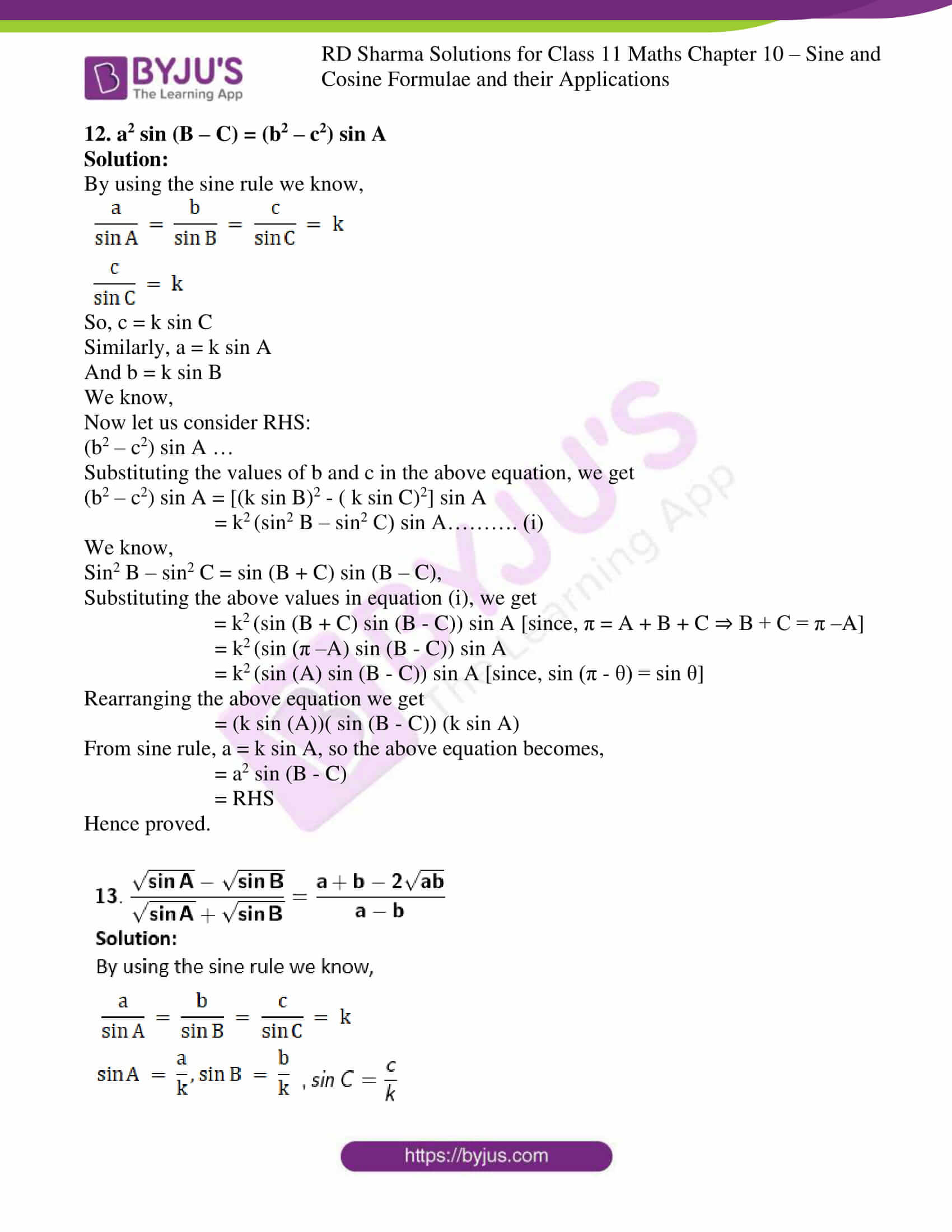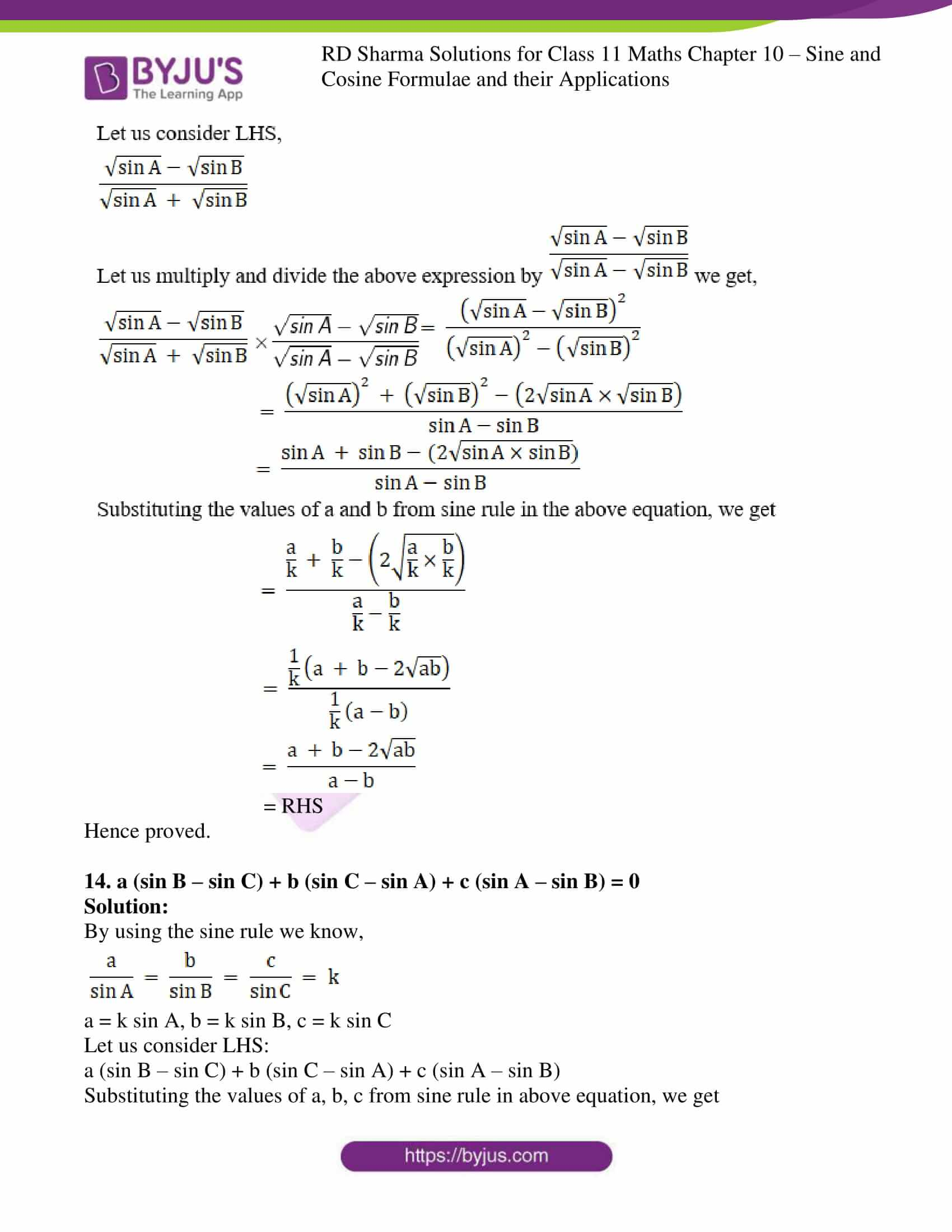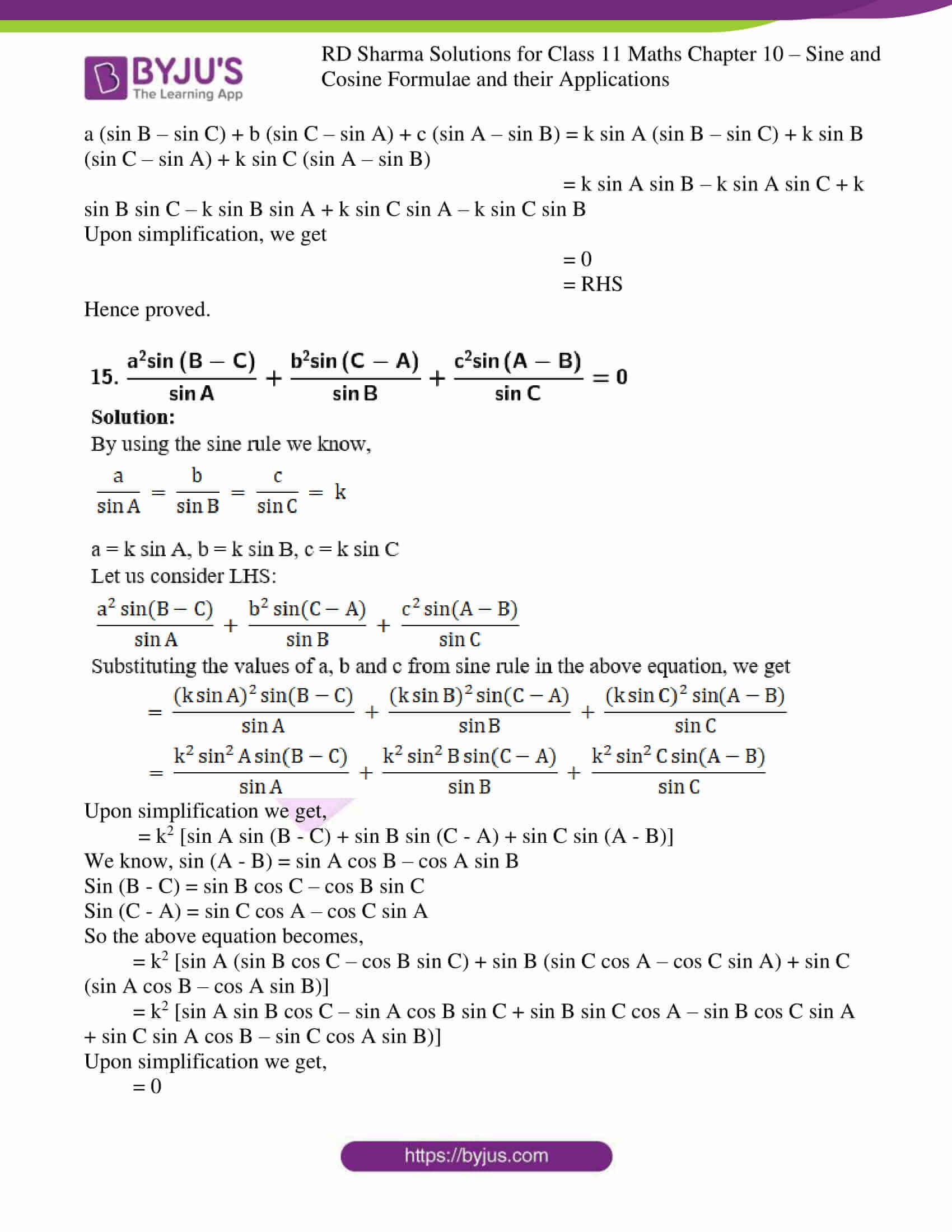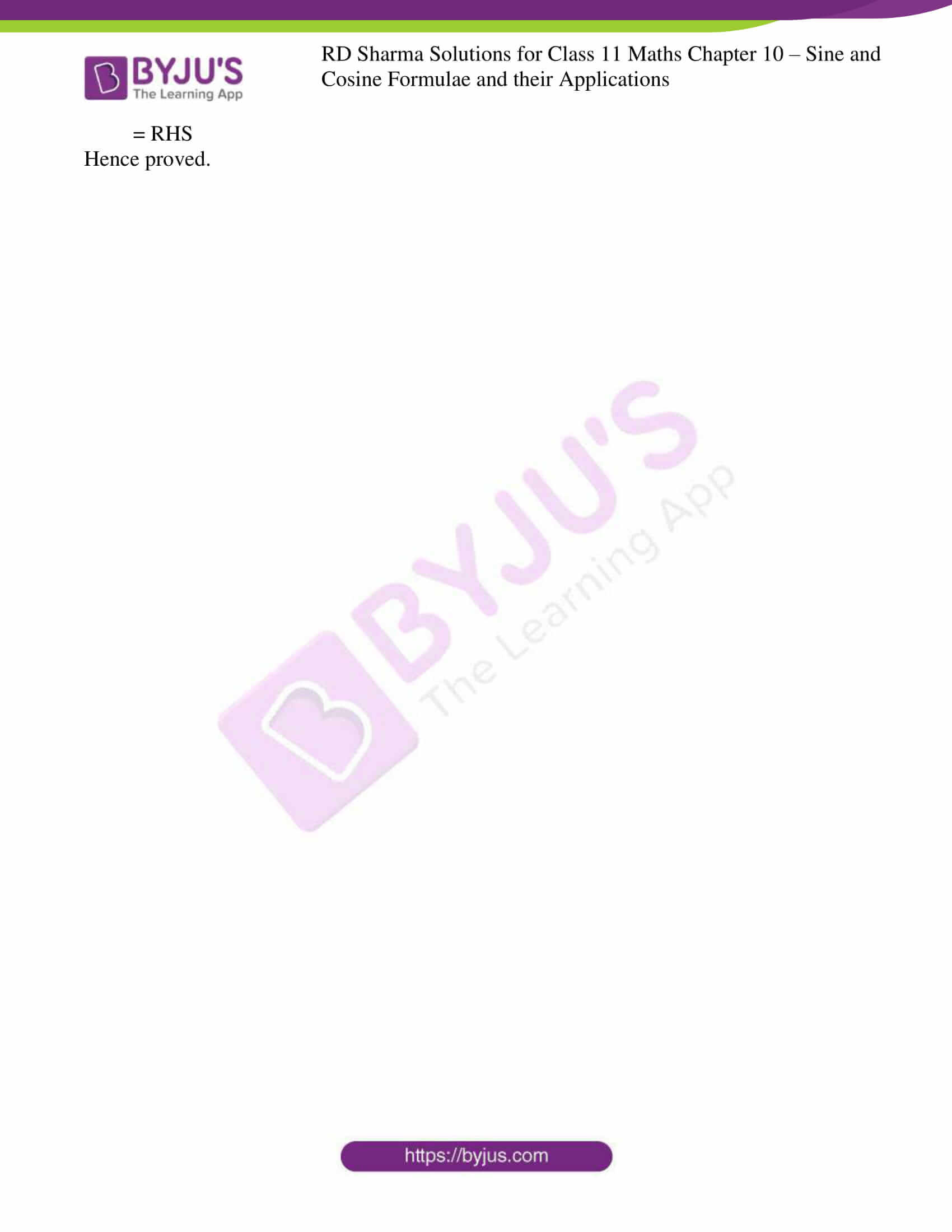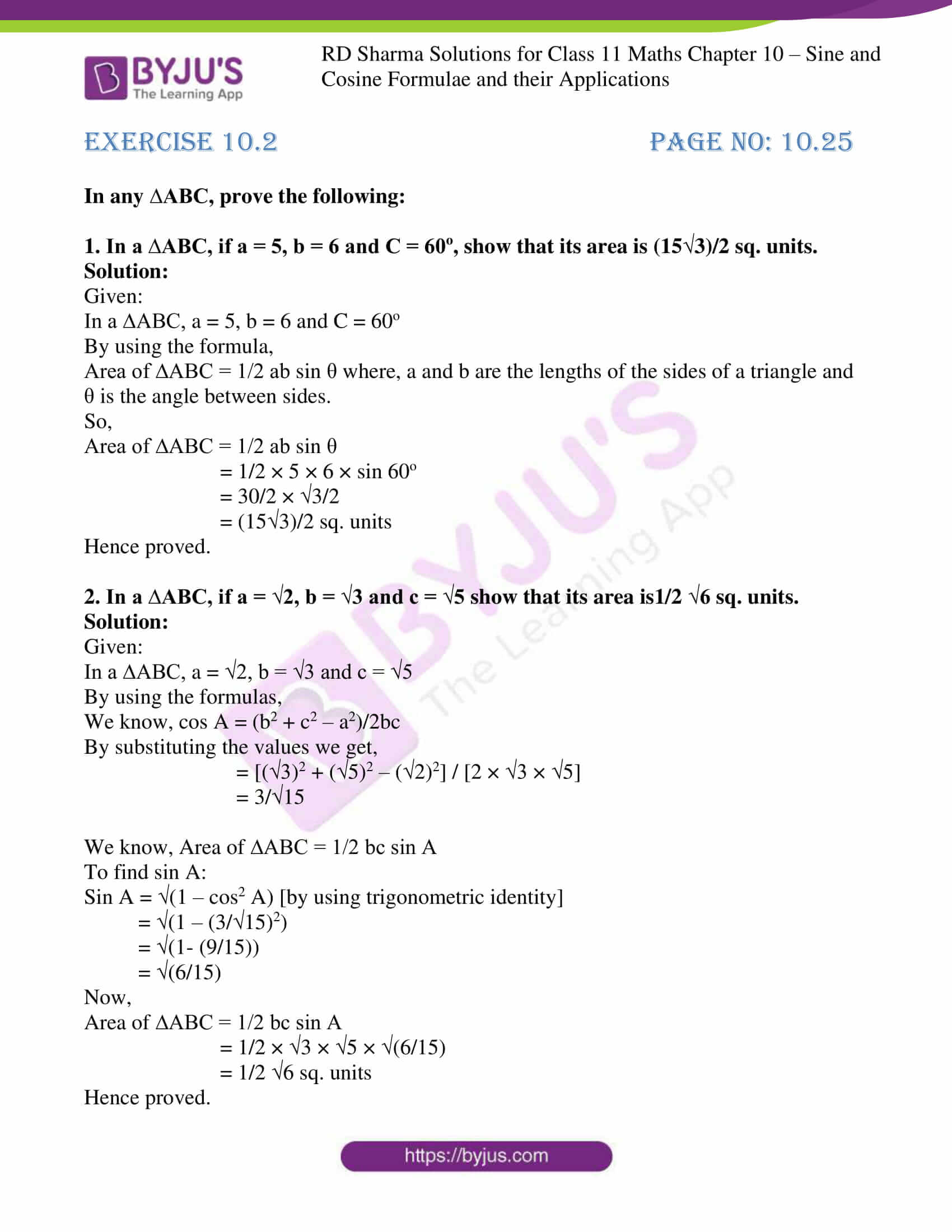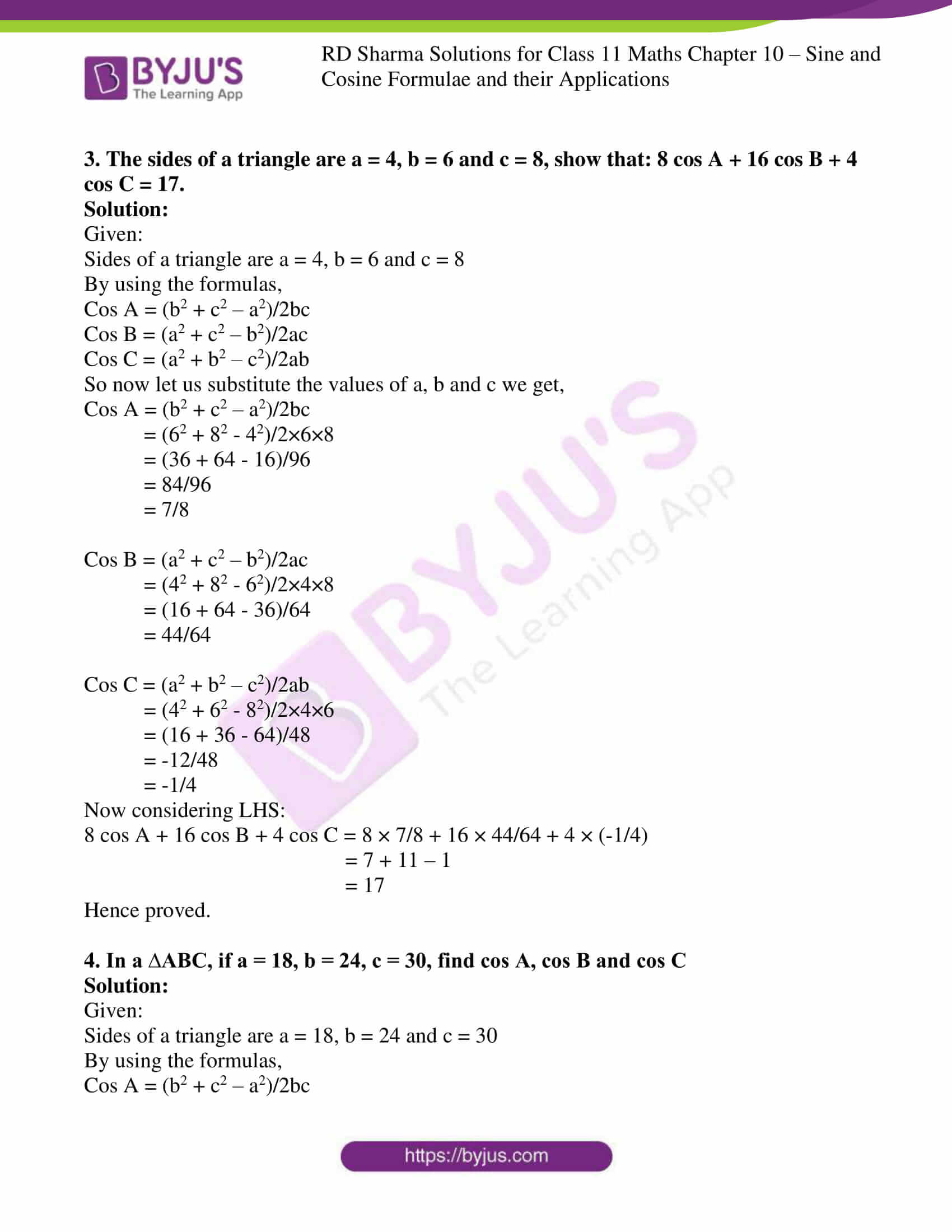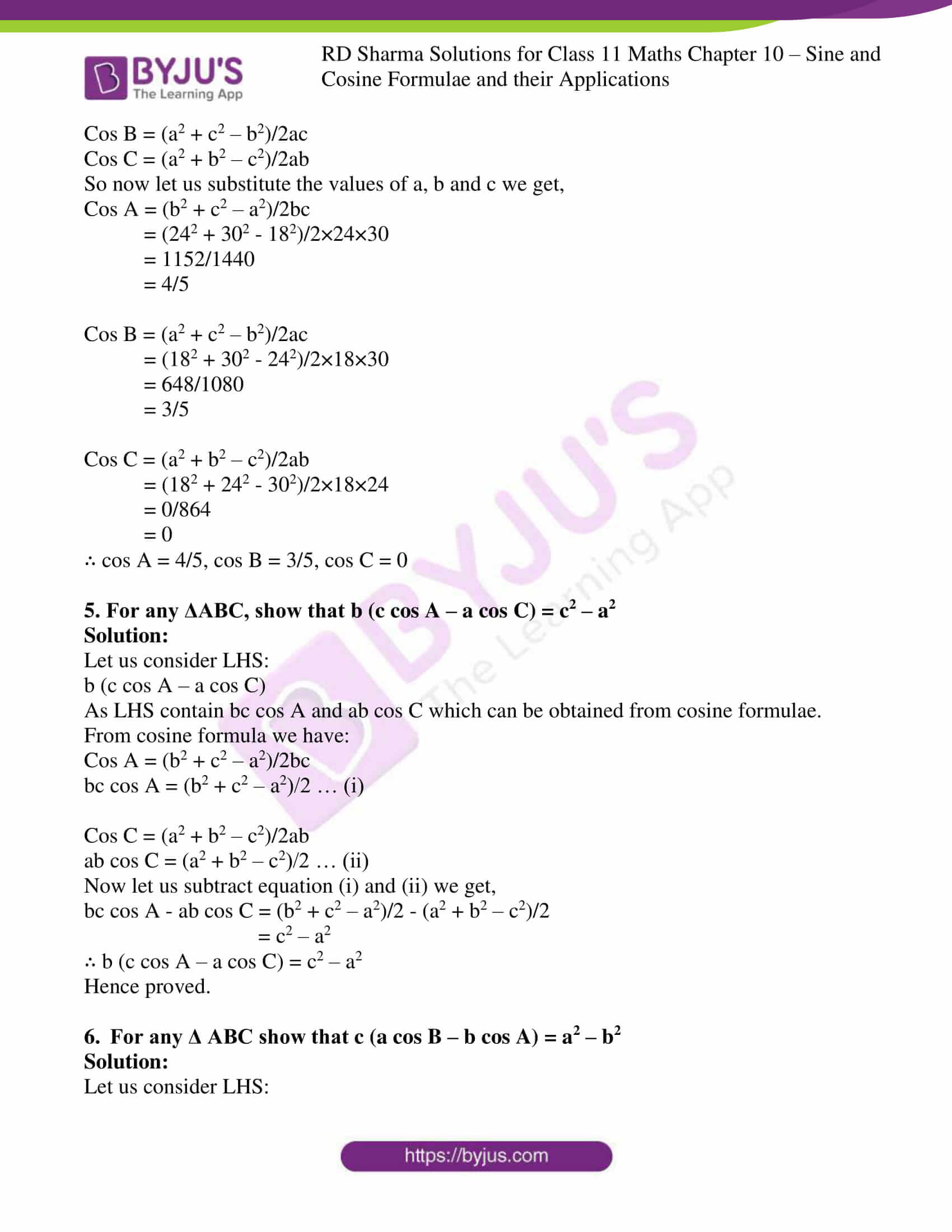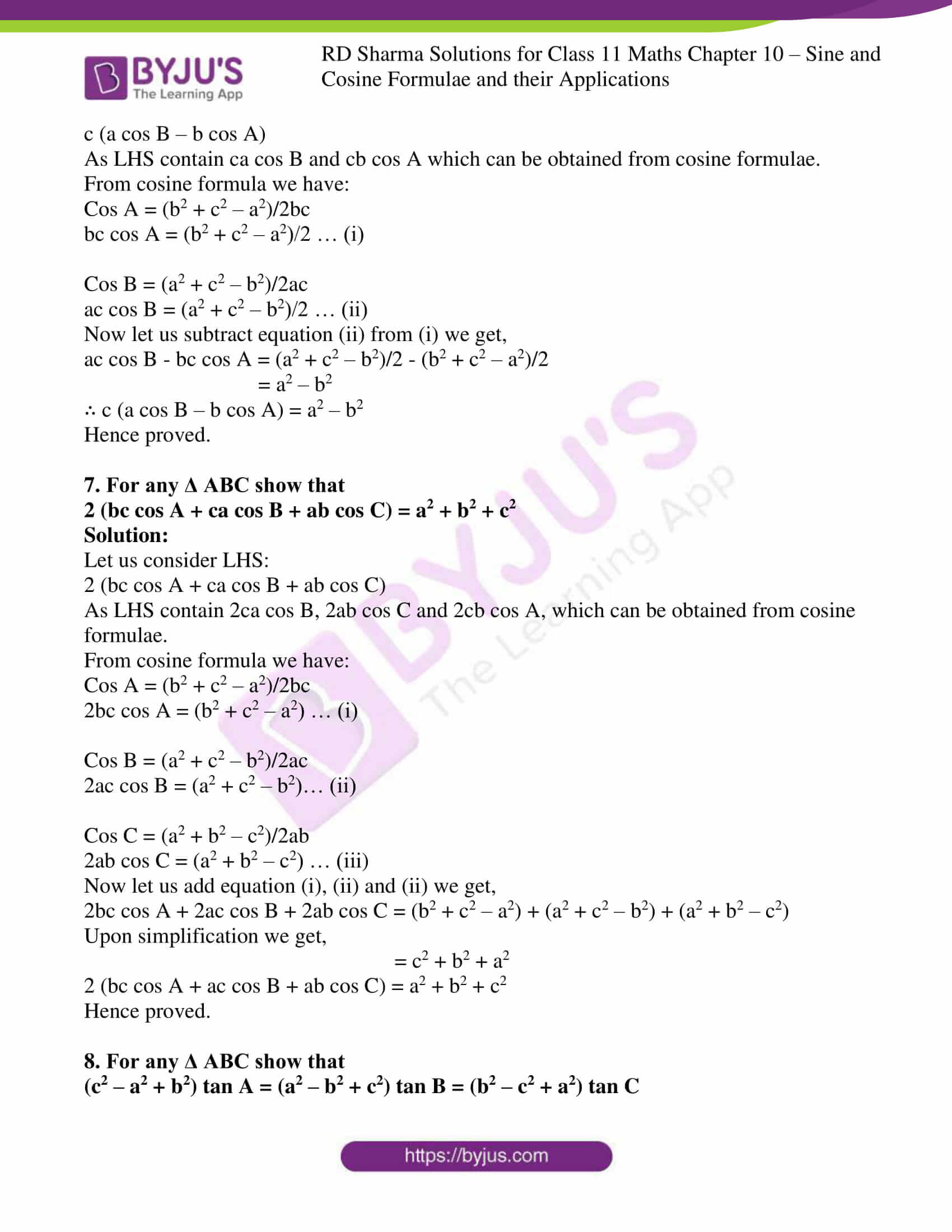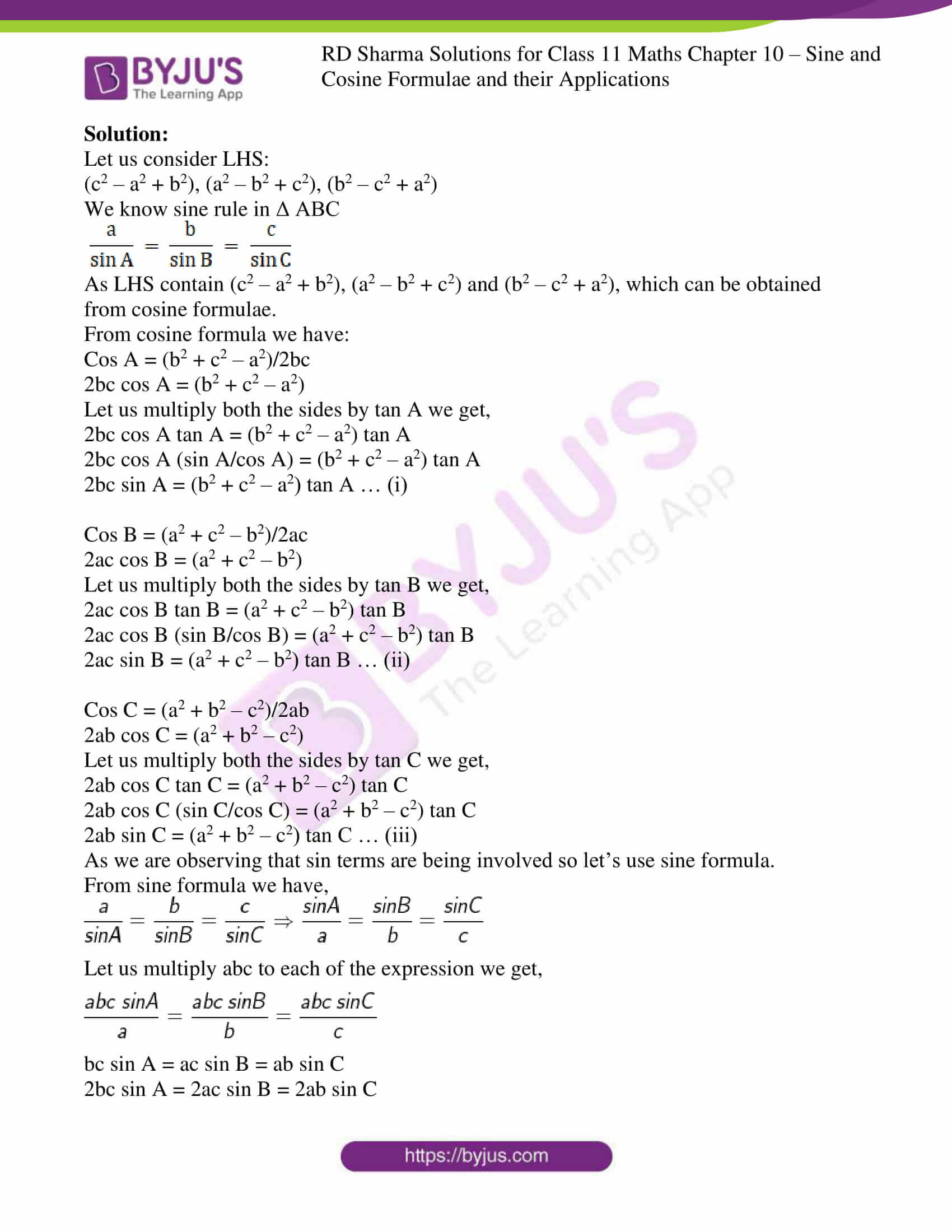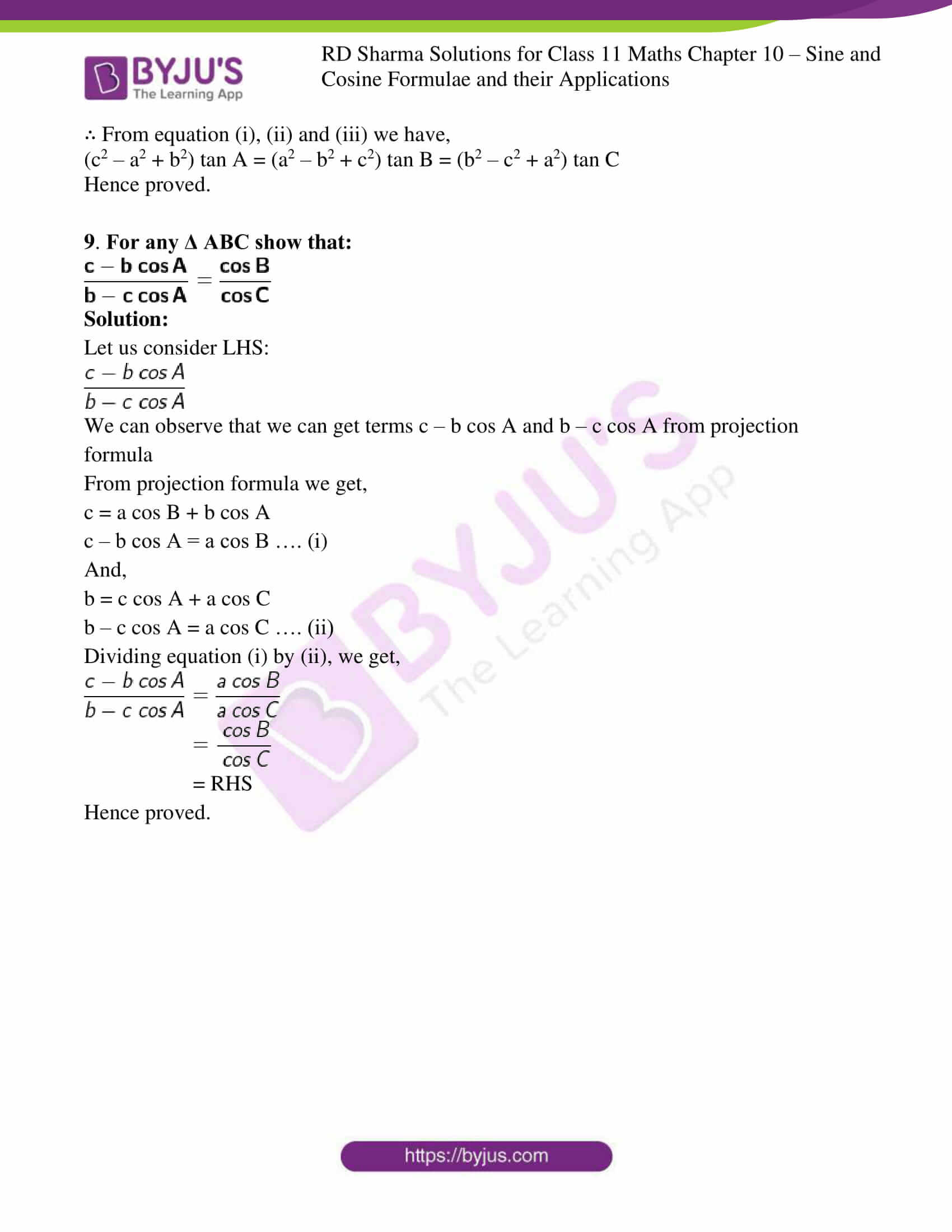### Also, access exercises of RD Sharma Solutions for Class 11 Maths Chapter 10 – Sine and Cosine Formulae and their Applications

Exercise 10.1 Solutions

Exercise 10.2 Solutions

### Access answers to RD Sharma Solutions for Class 11 Maths Chapter 10 – Sine and Cosine Formulae and their Applications

EXERCISE 10.1 PAGE NO: 10.12

1. If in a ∆ABC, ∠A = 45o, ∠B = 60o, and ∠C = 75o; find the ratio of its sides.

Solution:

Given: In ABC, ∠A = 45o, ∠B = 60o, and ∠C = 75o

By using the sine rule, we get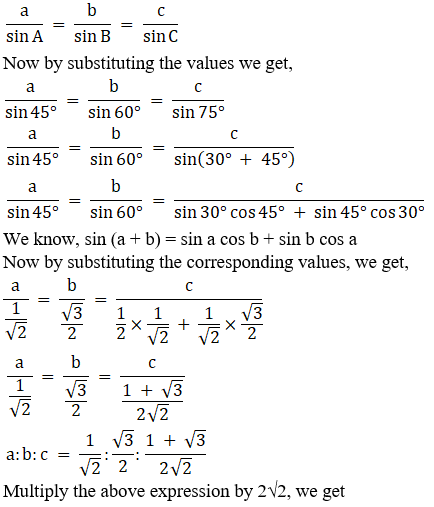a: b: c = 2: √6: (1+√3)

Hence the ratio of the sides of the given triangle is a: b: c = 2: √6: (1+√3)

2. If in any ∆ABC, ∠C = 105o, ∠B = 45o, a = 2, then find b.

Solution:

Given: In ∆ABC, ∠C = 105o, ∠B = 45o, a = 2

We know in a triangle,

∠A + ∠B + ∠C = 180°

∠A = 180° – ∠B – ∠C

Substituting the given values, we get

∠A = 180° – 45° – 105°

∠A = 30°

By using the sine rule, we get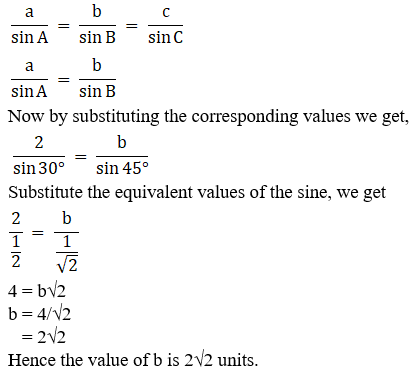3. In ∆ABC, if a = 18, b = 24 and c = 30 and ∠C = 90o, find sin A, sin B and sin C.

Solution:

Given: In ∆ABC, a = 18, b = 24 and c = 30 and ∠C = 90o

By using the sine rule, we get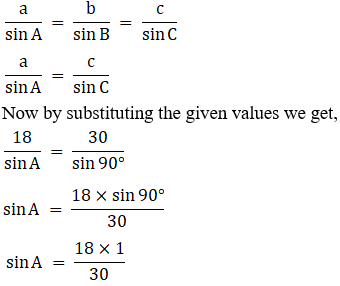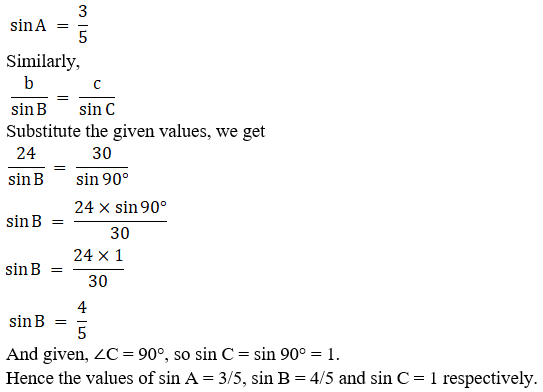In any triangle ABC, prove the following: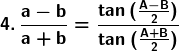Solution:

By using the sine rule we know,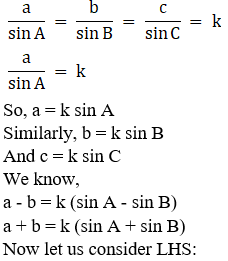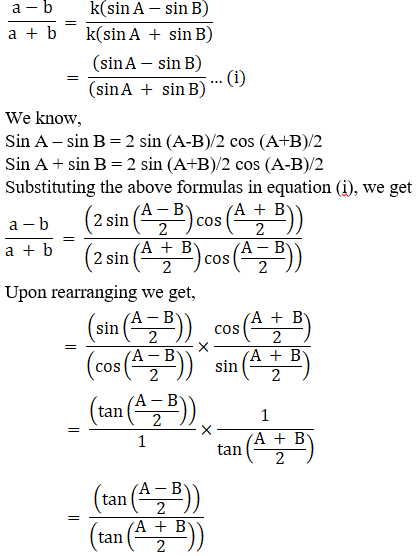= RHS

Hence proved.

5. (a – b) cos C/2 = C sin (A – B)/2

Solution:

By using the sine rule we know,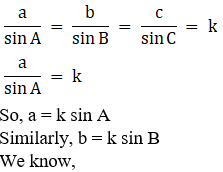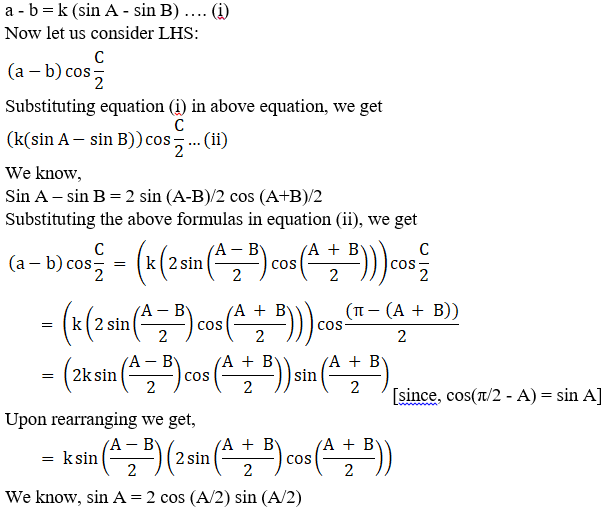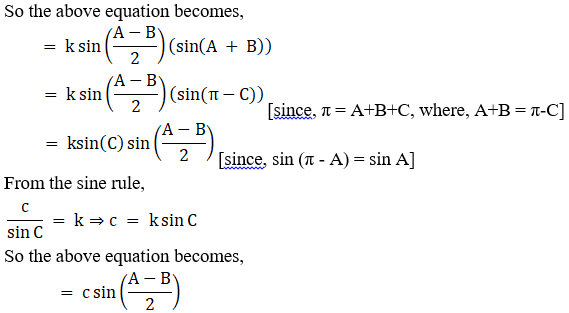= RHS

Hence proved.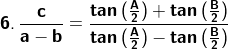Solution:

By using the sine rule we know,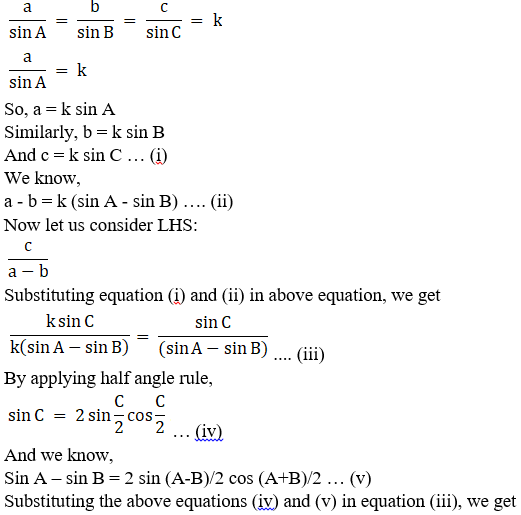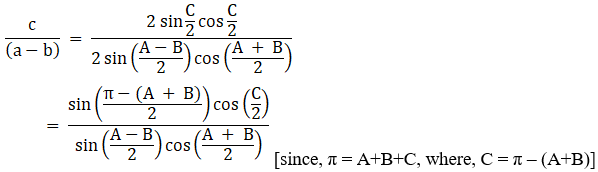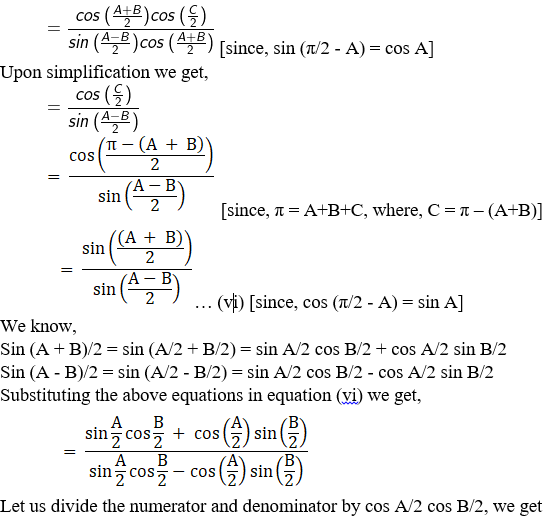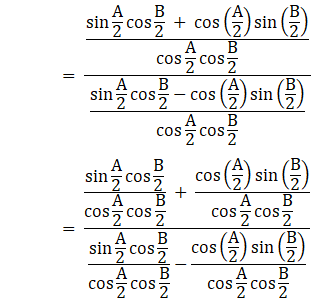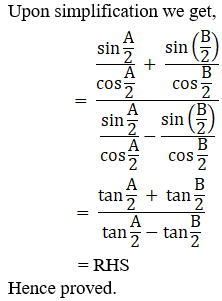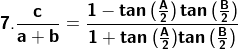Solution:

By using the sine rule we know,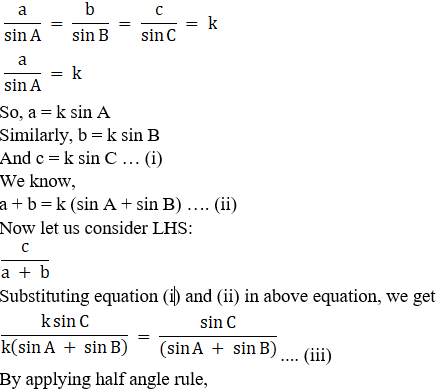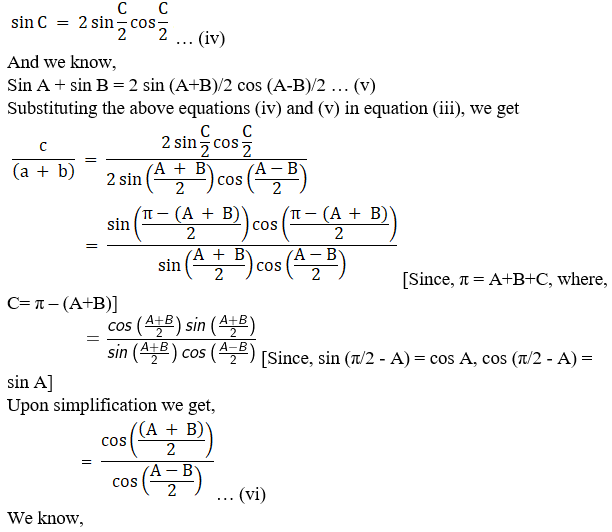cos (A + B)/2 = cos (A/2 + B/2) = cos A/2 cos B/2 + sin A/2 sin B/2

cos (A – B)/2 = cos (A/2 – B/2) = cos A/2 cos B/2 – sin A/2 sin B/2

Substituting the above equations in equation (vi) we get,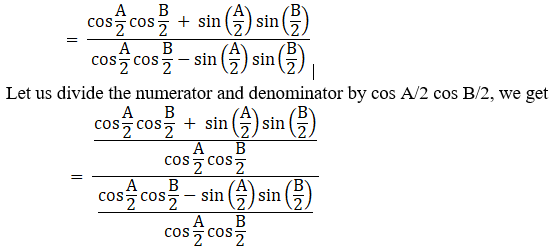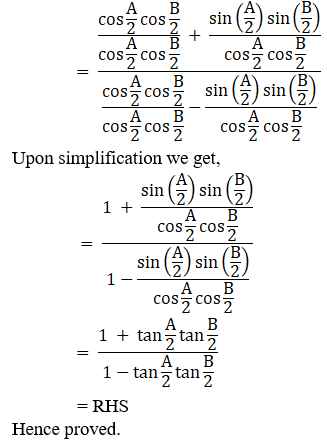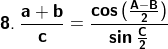Solution:

By using the sine rule we know,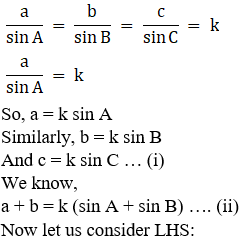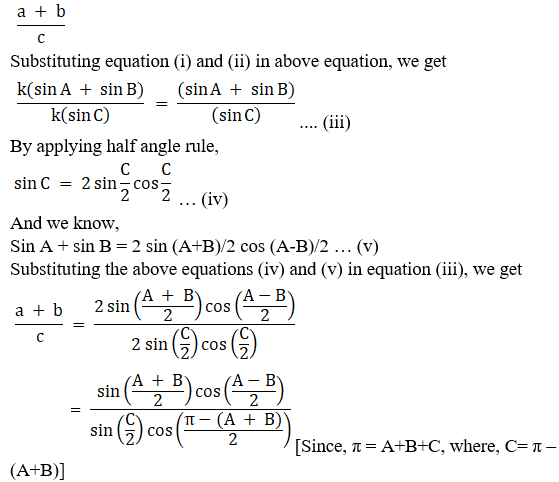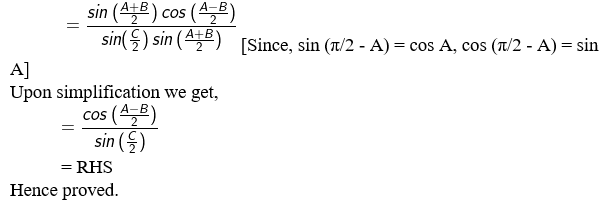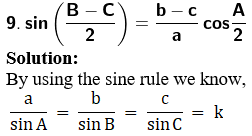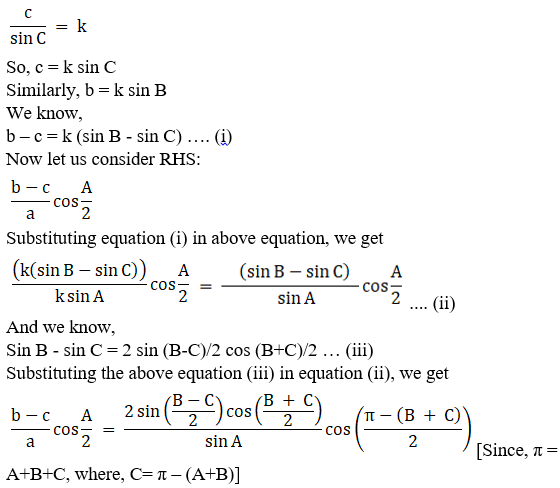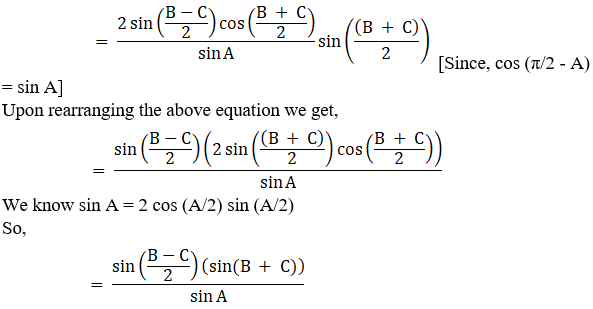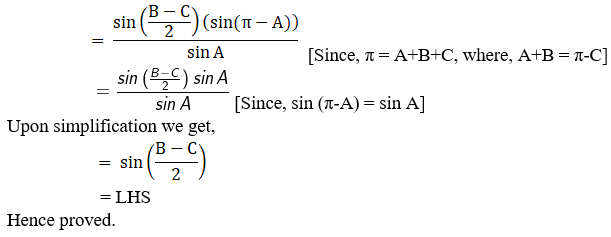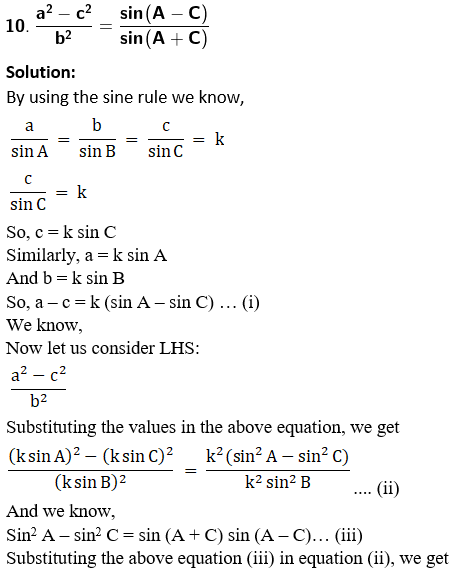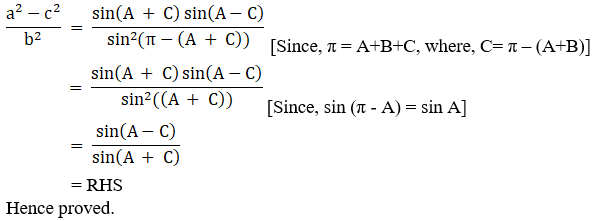11. b sin B – c sin C = a sin (B – C)

Solution:

By using the sine rule we know,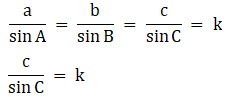So, c = k sin C

Similarly, a = k sin A

And b = k sin B

We know,

Now let us consider LHS:

b sin B – c sin C

Substituting the values of b and c in the above equation, we get

k sin B sin B – k sin C sin C = k (sin2 B – sin2 C) ……….(i)

We know,

Sin2 B – sin2 C = sin (B + C) sin (B – C),

Substituting the above values in equation (i), we get

k (sin2 B – sin2 C) = k (sin (B + C) sin (B – C)) [since, π = A + B + C ⇒ B + C = π –A]

The above equation becomes,

= k (sin (π –A) sin (B – C)) [since, sin (π – θ) = sin θ]

= k (sin (A) sin (B – C))

From sine rule, a = k sin A, so the above equation becomes,

= a sin (B – C)

= RHS

Hence proved.

12. a2 sin (B – C) = (b2 – c2) sin A

Solution:

By using the sine rule we know,So, c = k sin C

Similarly, a = k sin A

And b = k sin B

We know,

Now let us consider RHS:

(b2 – c2) sin A …

Substituting the values of b and c in the above equation, we get

(b2 – c2) sin A = [(k sin B)2 – ( k sin C)2] sin A

= k2 (sin2 B – sin2 C) sin A………. (i)

We know,

Sin2 B – sin2 C = sin (B + C) sin (B – C),

Substituting the above values in equation (i), we get

= k2 (sin (B + C) sin (B – C)) sin A [since, π = A + B + C ⇒ B + C = π –A]

= k2 (sin (π –A) sin (B – C)) sin A

= k2 (sin (A) sin (B – C)) sin A [since, sin (π – θ) = sin θ]

Rearranging the above equation we get

= (k sin (A))( sin (B – C)) (k sin A)

From sine rule, a = k sin A, so the above equation becomes,

= a2 sin (B – C)

= RHS

Hence proved.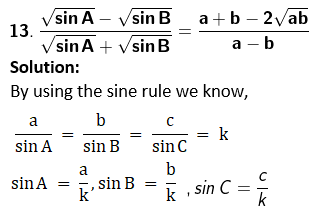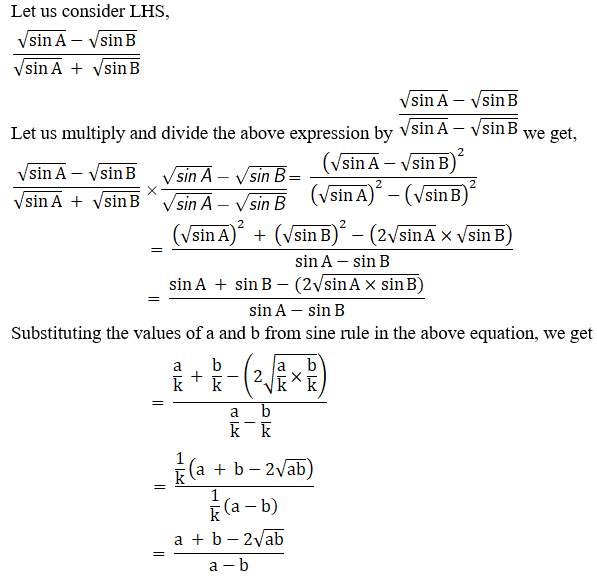= RHS

Hence proved.

14. a (sin B – sin C) + b (sin C – sin A) + c (sin A – sin B) = 0

Solution:

By using the sine rule we know,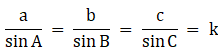a = k sin A, b = k sin B, c = k sin C

Let us consider LHS:

a (sin B – sin C) + b (sin C – sin A) + c (sin A – sin B)

Substituting the values of a, b, c from sine rule in above equation, we get

a (sin B – sin C) + b (sin C – sin A) + c (sin A – sin B) = k sin A (sin B – sin C) + k sin B (sin C – sin A) + k sin C (sin A – sin B)

= k sin A sin B – k sin A sin C + k sin B sin C – k sin B sin A + k sin C sin A – k sin C sin B

Upon simplification, we get

= 0

= RHS

Hence proved.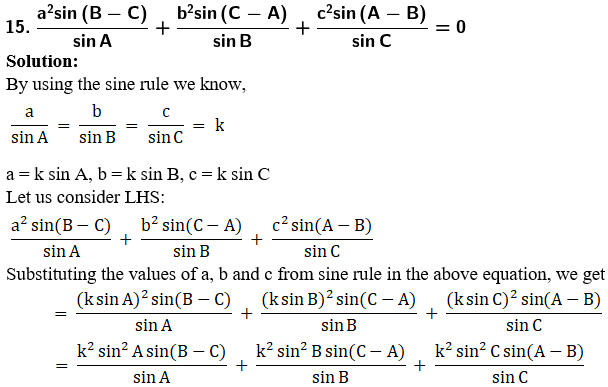Upon simplification we get,

= k2 [sin A sin (B – C) + sin B sin (C – A) + sin C sin (A – B)]

We know, sin (A – B) = sin A cos B – cos A sin B

Sin (B – C) = sin B cos C – cos B sin C

Sin (C – A) = sin C cos A – cos C sin A

So the above equation becomes,

= k2 [sin A (sin B cos C – cos B sin C) + sin B (sin C cos A – cos C sin A) + sin C (sin A cos B – cos A sin B)]

= k2 [sin A sin B cos C – sin A cos B sin C + sin B sin C cos A – sin B cos C sin A + sin C sin A cos B – sin C cos A sin B)]

Upon simplification we get,

= 0

= RHS

Hence proved.

EXERCISE 10.2 PAGE NO: 10.25

In any ∆ABC, prove the following:

1. In a ∆ABC, if a = 5, b = 6 and C = 60o, show that its area is (15√3)/2 sq. units.

Solution:

Given:

In a ∆ABC, a = 5, b = 6 and C = 60o

By using the formula,

Area of ∆ABC = 1/2 ab sin θ where, a and b are the lengths of the sides of a triangle and θ is the angle between sides.

So,

Area of ∆ABC = 1/2 ab sin θ

= 1/2 × 5 × 6 × sin 60o

= 30/2 × 3/2

= (153)/2 sq. units

Hence proved.

2. In a ∆ABC, if a = √2, b = √3 and c = √5 show that its area is1/2 √6 sq. units.

Solution:

Given:

In a ∆ABC, a = √2, b = √3 and c = √5

By using the formulas,

We know, cos A = (b2 + c2 – a2)/2bc

By substituting the values we get,

= [(√3)2 + (√5)2 – (√2)2] / [2 × √3 × √5]

= 3/√15

We know, Area of ∆ABC = 1/2 bc sin A

To find sin A:

Sin A = √(1 – cos2 A) [by using trigonometric identity]

= √(1 – (3/√15)2)

= √(1- (9/15))

= √(6/15)

Now,

Area of ∆ABC = 1/2 bc sin A

= 1/2 × √3 × √5 × √(6/15)

= 1/2 √6 sq. units

Hence proved.

3. The sides of a triangle are a = 4, b = 6 and c = 8, show that: 8 cos A + 16 cos B + 4 cos C = 17.

Solution:

Given:

Sides of a triangle are a = 4, b = 6 and c = 8

By using the formulas,

Cos A = (b2 + c2 – a2)/2bc

Cos B = (a2 + c2 – b2)/2ac

Cos C = (a2 + b2 – c2)/2ab

So now let us substitute the values of a, b and c we get,

Cos A = (b2 + c2 – a2)/2bc

= (62 + 82 – 42)/2×6×8

= (36 + 64 – 16)/96

= 84/96

= 7/8

Cos B = (a2 + c2 – b2)/2ac

= (42 + 82 – 62)/2×4×8

= (16 + 64 – 36)/64

= 44/64

Cos C = (a2 + b2 – c2)/2ab

= (42 + 62 – 82)/2×4×6

= (16 + 36 – 64)/48

= -12/48

= -1/4

Now considering LHS:

8 cos A + 16 cos B + 4 cos C = 8 × 7/8 + 16 × 44/64 + 4 × (-1/4)

= 7 + 11 – 1

= 17

Hence proved.

4. In a ∆ABC, if a = 18, b = 24, c = 30, find cos A, cos B and cos C

Solution:

Given:

Sides of a triangle are a = 18, b = 24 and c = 30

By using the formulas,

Cos A = (b2 + c2 – a2)/2bc

Cos B = (a2 + c2 – b2)/2ac

Cos C = (a2 + b2 – c2)/2ab

So now let us substitute the values of a, b and c we get,

Cos A = (b2 + c2 – a2)/2bc

= (242 + 302 – 182)/2×24×30

= 1152/1440

= 4/5

Cos B = (a2 + c2 – b2)/2ac

= (182 + 302 – 242)/2×18×30

= 648/1080

= 3/5

Cos C = (a2 + b2 – c2)/2ab

= (182 + 242 – 302)/2×18×24

= 0/864

= 0

∴ cos A = 4/5, cos B = 3/5, cos C = 0

5. For any ΔABC, show that b (c cos A – a cos C) = c2 – a2

Solution:

Let us consider LHS:

b (c cos A – a cos C)

As LHS contain bc cos A and ab cos C which can be obtained from cosine formulae.

From cosine formula we have:

Cos A = (b2 + c2 – a2)/2bc

bc cos A = (b2 + c2 – a2)/2 … (i)

Cos C = (a2 + b2 – c2)/2ab

ab cos C = (a2 + b2 – c2)/2 … (ii)

Now let us subtract equation (i) and (ii) we get,

bc cos A – ab cos C = (b2 + c2 – a2)/2 – (a2 + b2 – c2)/2

= c2 – a2

∴ b (c cos A – a cos C) = c2 – a2

Hence proved.

6. For any Δ ABC show that c (a cos B – b cos A) = a2 – b2

Solution:

Let us consider LHS:

c (a cos B – b cos A)

As LHS contain ca cos B and cb cos A which can be obtained from cosine formulae.

From cosine formula we have:

Cos A = (b2 + c2 – a2)/2bc

bc cos A = (b2 + c2 – a2)/2 … (i)

Cos B = (a2 + c2 – b2)/2ac

ac cos B = (a2 + c2 – b2)/2 … (ii)

Now let us subtract equation (ii) from (i) we get,

ac cos B – bc cos A = (a2 + c2 – b2)/2 – (b2 + c2 – a2)/2

= a2 – b2

∴ c (a cos B – b cos A) = a2 – b2

Hence proved.

7. For any Δ ABC show that
2 (bc cos A + ca cos B + ab cos C) = a2 + b2 + c2

Solution:

Let us consider LHS:

2 (bc cos A + ca cos B + ab cos C)

As LHS contain 2ca cos B, 2ab cos C and 2cb cos A, which can be obtained from cosine formulae.

From cosine formula we have:

Cos A = (b2 + c2 – a2)/2bc

2bc cos A = (b2 + c2 – a2) … (i)

Cos B = (a2 + c2 – b2)/2ac

2ac cos B = (a2 + c2 – b2)… (ii)

Cos C = (a2 + b2 – c2)/2ab

2ab cos C = (a2 + b2 – c2) … (iii)

Now let us add equation (i), (ii) and (ii) we get,

2bc cos A + 2ac cos B + 2ab cos C = (b2 + c2 – a2) + (a2 + c2 – b2) + (a2 + b2 – c2)

Upon simplification we get,

= c2 + b2 + a2

2 (bc cos A + ac cos B + ab cos C) = a2 + b2 + c2

Hence proved.

8. For any Δ ABC show that
(c2 – a2 + b2) tan A = (a2 – b2 + c2) tan B = (b2 – c2 + a2) tan C

Solution:

Let us consider LHS:

(c2 – a2 + b2), (a2 – b2 + c2), (b2 – c2 + a2)

We know sine rule in Δ ABC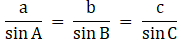As LHS contain (c2 – a2 + b2), (a2 – b2 + c2) and (b2 – c2 + a2), which can be obtained from cosine formulae.

From cosine formula we have:

Cos A = (b2 + c2 – a2)/2bc

2bc cos A = (b2 + c2 – a2)

Let us multiply both the sides by tan A we get,

2bc cos A tan A = (b2 + c2 – a2) tan A

2bc cos A (sin A/cos A) = (b2 + c2 – a2) tan A

2bc sin A = (b2 + c2 – a2) tan A … (i)

Cos B = (a2 + c2 – b2)/2ac

2ac cos B = (a2 + c2 – b2)

Let us multiply both the sides by tan B we get,

2ac cos B tan B = (a2 + c2 – b2) tan B

2ac cos B (sin B/cos B) = (a2 + c2 – b2) tan B

2ac sin B = (a2 + c2 – b2) tan B … (ii)

Cos C = (a2 + b2 – c2)/2ab

2ab cos C = (a2 + b2 – c2)

Let us multiply both the sides by tan C we get,

2ab cos C tan C = (a2 + b2 – c2) tan C

2ab cos C (sin C/cos C) = (a2 + b2 – c2) tan C

2ab sin C = (a2 + b2 – c2) tan C … (iii)

As we are observing that sin terms are being involved so let’s use sine formula.

From sine formula we have,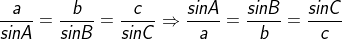Let us multiply abc to each of the expression we get,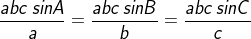bc sin A = ac sin B = ab sin C

2bc sin A = 2ac sin B = 2ab sin C

∴ From equation (i), (ii) and (iii) we have,

(c2 – a2 + b2) tan A = (a2 – b2 + c2) tan B = (b2 – c2 + a2) tan C

Hence proved.

9. For any Δ ABC show that: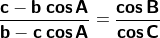Solution:

Let us consider LHS: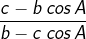We can observe that we can get terms c – b cos A and b – c cos A from projection formula

From projection formula we get,

c = a cos B + b cos A

c – b cos A = a cos B …. (i)

And,

b = c cos A + a cos C

b – c cos A = a cos C …. (ii)

Dividing equation (i) by (ii), we get,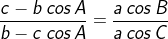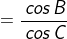= RHS

Hence proved.

## Frequently Asked Questions on RD Sharma Solutions for Class 11 Maths Chapter 10

### Does the RD Sharma Solutions for Class 11 Maths Chapter 10 PDF help students for exam preparations?

Yes, the RD Sharma Solutions for Class 11 Maths Chapter 10 PDFs consists of solutions to the textbook questions presented in a detailed manner highlighting the main topics which help students in their exam preparation. The answers in the solutions PDF are written by a highly experienced faculty team of members having vast experience in the subject at BYJU’S as per the CBSE guidelines and exam patterns. Students can comprehend the key topics better and score well in the board exams with this resource. Further, the solutions PDFs are available for free download and can be used while learning to answer the questions from the textbook.

### How can I effectively use the RD Sharma Solutions for Class 11 Maths Chapter 10?

Students can strongly rely on RD Sharma Solutions for Class 11 Maths Chapter 10 resources for exam preparations since subject experts at BYJU’S have formulated all the answers to the textbook questions. By practising the solutions provided in the solutions PDF, students will be able to write precise and elaborate answers to the questions by following the latest CBSE guidelines.

### Is RD Sharma Solutions for Class 11 Maths Chapter 10 available in PDF format at BYJU’S?

Yes, BYJU’S provides the RD Sharma Solutions for Class 11 Maths Chapter 10 in PDF format which can be downloaded by the students. The PDF’s created by the experts are of high quality and the free download links can be used by the students to access them. The reference materials from BYJU’S are hassle free and help students to score well in the board exams.